# JP4764939B2 - Recording condition adjusting method and optical disc apparatus - Google Patents

## Info

Publication number
JP4764939B2
JP4764939B2 JP2009097590A JP2009097590A JP4764939B2 JP 4764939 B2 JP4764939 B2 JP 4764939B2 JP 2009097590 A JP2009097590 A JP 2009097590A JP 2009097590 A JP2009097590 A JP 2009097590A JP 4764939 B2 JP4764939 B2 JP 4764939B2
Authority
JP
Japan
Prior art keywords
bit string
edge
recording
evaluation
signal waveform
Prior art date
Legal status (The legal status is an assumption and is not a legal conclusion. Google has not performed a legal analysis and makes no representation as to the accuracy of the status listed.)
Active
Application number
JP2009097590A
Other languages
Japanese (ja)
Other versions
JP2010250881A (en
Inventor

Original Assignee

Priority date (The priority date is an assumption and is not a legal conclusion. Google has not performed a legal analysis and makes no representation as to the accuracy of the date listed.)
Filing date
Publication date
Application filed by 株式会社日立製作所 filed Critical 株式会社日立製作所
Priority to JP2009097590A priority Critical patent/JP4764939B2/en
Publication of JP2010250881A publication Critical patent/JP2010250881A/en
Application granted granted Critical
Publication of JP4764939B2 publication Critical patent/JP4764939B2/en
Active legal-status Critical Current
Anticipated expiration legal-status Critical

• 230000003287 optical Effects 0.000 title claims description 75
• 230000000875 corresponding Effects 0.000 claims description 53
• 230000003044 adaptive Effects 0.000 claims description 22
• 238000000034 methods Methods 0.000 description 25
• 238000004088 simulation Methods 0.000 description 19
• 238000004364 calculation methods Methods 0.000 description 10
• 230000000694 effects Effects 0.000 description 8
• 239000000203 mixtures Substances 0.000 description 7
• 238000005070 sampling Methods 0.000 description 4
• 239000010408 films Substances 0.000 description 3
• 238000006243 chemical reaction Methods 0.000 description 2
• 239000000463 materials Substances 0.000 description 2
• 239000000956 alloys Substances 0.000 description 1
• 238000004519 manufacturing process Methods 0.000 description 1
• 239000002184 metal Substances 0.000 description 1
• 230000000704 physical effects Effects 0.000 description 1

## Images

•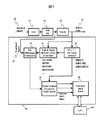•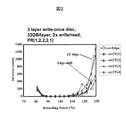•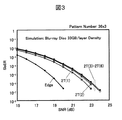•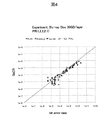•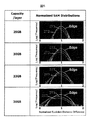•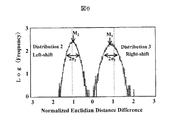•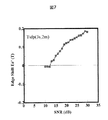•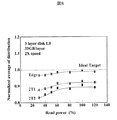•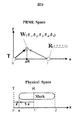••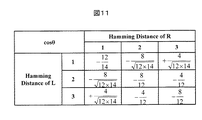•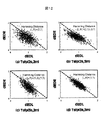•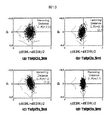•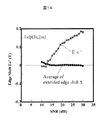•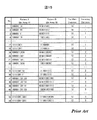•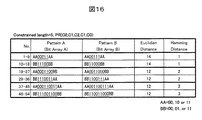••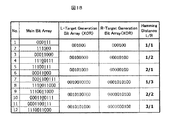•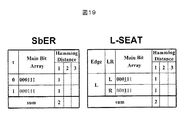•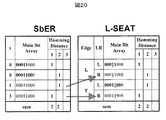•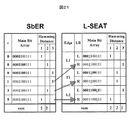•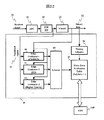•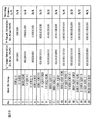•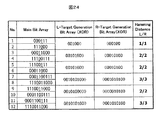•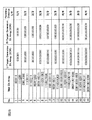•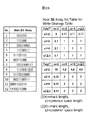•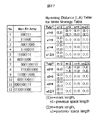•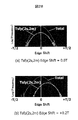•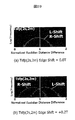•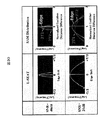••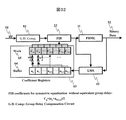•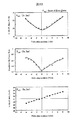•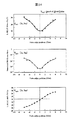•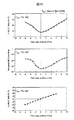•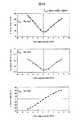••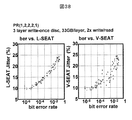•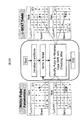•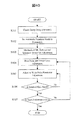•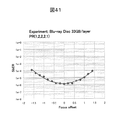•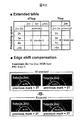•## Classifications

• GPHYSICS
• G11INFORMATION STORAGE
• G11BINFORMATION STORAGE BASED ON RELATIVE MOVEMENT BETWEEN RECORD CARRIER AND TRANSDUCER
• G11B20/00Signal processing not specific to the method of recording or reproducing; Circuits therefor
• G11B20/10Digital recording or reproducing
• G11B20/10009Improvement or modification of read or write signals
• GPHYSICS
• G11INFORMATION STORAGE
• G11BINFORMATION STORAGE BASED ON RELATIVE MOVEMENT BETWEEN RECORD CARRIER AND TRANSDUCER
• G11B20/00Signal processing not specific to the method of recording or reproducing; Circuits therefor
• G11B20/10Digital recording or reproducing
• G11B20/10009Improvement or modification of read or write signals
• G11B20/10046Improvement or modification of read or write signals filtering or equalising, e.g. setting the tap weights of an FIR filter
• GPHYSICS
• G11INFORMATION STORAGE
• G11BINFORMATION STORAGE BASED ON RELATIVE MOVEMENT BETWEEN RECORD CARRIER AND TRANSDUCER
• G11B20/00Signal processing not specific to the method of recording or reproducing; Circuits therefor
• G11B20/10Digital recording or reproducing
• G11B20/10009Improvement or modification of read or write signals
• G11B20/10046Improvement or modification of read or write signals filtering or equalising, e.g. setting the tap weights of an FIR filter
• G11B20/10055Improvement or modification of read or write signals filtering or equalising, e.g. setting the tap weights of an FIR filter using partial response filtering when writing the signal to the medium or reading it therefrom
• GPHYSICS
• G11INFORMATION STORAGE
• G11BINFORMATION STORAGE BASED ON RELATIVE MOVEMENT BETWEEN RECORD CARRIER AND TRANSDUCER
• G11B20/00Signal processing not specific to the method of recording or reproducing; Circuits therefor
• G11B20/10Digital recording or reproducing
• G11B20/10009Improvement or modification of read or write signals
• G11B20/10046Improvement or modification of read or write signals filtering or equalising, e.g. setting the tap weights of an FIR filter
• G11B20/10055Improvement or modification of read or write signals filtering or equalising, e.g. setting the tap weights of an FIR filter using partial response filtering when writing the signal to the medium or reading it therefrom
• G11B20/1012Improvement or modification of read or write signals filtering or equalising, e.g. setting the tap weights of an FIR filter using partial response filtering when writing the signal to the medium or reading it therefrom partial response PR(1,2,2,2,1)
• GPHYSICS
• G11INFORMATION STORAGE
• G11BINFORMATION STORAGE BASED ON RELATIVE MOVEMENT BETWEEN RECORD CARRIER AND TRANSDUCER
• G11B20/00Signal processing not specific to the method of recording or reproducing; Circuits therefor
• G11B20/10Digital recording or reproducing
• G11B20/10009Improvement or modification of read or write signals
• G11B20/10046Improvement or modification of read or write signals filtering or equalising, e.g. setting the tap weights of an FIR filter
• G11B20/10212Improvement or modification of read or write signals filtering or equalising, e.g. setting the tap weights of an FIR filter compensation for data shift (e.g. pulse crowding effects)
• GPHYSICS
• G11INFORMATION STORAGE
• G11BINFORMATION STORAGE BASED ON RELATIVE MOVEMENT BETWEEN RECORD CARRIER AND TRANSDUCER
• G11B20/00Signal processing not specific to the method of recording or reproducing; Circuits therefor
• G11B20/10Digital recording or reproducing
• G11B20/10009Improvement or modification of read or write signals
• G11B20/10268Improvement or modification of read or write signals bit detection or demodulation methods
• G11B20/10287Improvement or modification of read or write signals bit detection or demodulation methods using probabilistic methods, e.g. maximum likelihood detectors
• G11B20/10296Improvement or modification of read or write signals bit detection or demodulation methods using probabilistic methods, e.g. maximum likelihood detectors using the Viterbi algorithm
• GPHYSICS
• G11INFORMATION STORAGE
• G11BINFORMATION STORAGE BASED ON RELATIVE MOVEMENT BETWEEN RECORD CARRIER AND TRANSDUCER
• G11B20/00Signal processing not specific to the method of recording or reproducing; Circuits therefor
• G11B20/10Digital recording or reproducing
• G11B20/10009Improvement or modification of read or write signals
• G11B20/10481Improvement or modification of read or write signals optimisation methods
• GPHYSICS
• G11INFORMATION STORAGE
• G11BINFORMATION STORAGE BASED ON RELATIVE MOVEMENT BETWEEN RECORD CARRIER AND TRANSDUCER
• G11B20/00Signal processing not specific to the method of recording or reproducing; Circuits therefor
• G11B20/10Digital recording or reproducing
• G11B20/18Error detection or correction; Testing, e.g. of drop-outs
• G11B20/1816Testing
• GPHYSICS
• G11INFORMATION STORAGE
• G11BINFORMATION STORAGE BASED ON RELATIVE MOVEMENT BETWEEN RECORD CARRIER AND TRANSDUCER
• G11B20/00Signal processing not specific to the method of recording or reproducing; Circuits therefor
• G11B20/10Digital recording or reproducing
• G11B20/18Error detection or correction; Testing, e.g. of drop-outs
• G11B20/1816Testing
• G11B20/182Testing using test patterns
• GPHYSICS
• G11INFORMATION STORAGE
• G11BINFORMATION STORAGE BASED ON RELATIVE MOVEMENT BETWEEN RECORD CARRIER AND TRANSDUCER
• G11B20/00Signal processing not specific to the method of recording or reproducing; Circuits therefor
• G11B20/22Signal processing not specific to the method of recording or reproducing; Circuits therefor for reducing distortions
• GPHYSICS
• G11INFORMATION STORAGE
• G11BINFORMATION STORAGE BASED ON RELATIVE MOVEMENT BETWEEN RECORD CARRIER AND TRANSDUCER
• G11B20/00Signal processing not specific to the method of recording or reproducing; Circuits therefor
• G11B20/22Signal processing not specific to the method of recording or reproducing; Circuits therefor for reducing distortions
• G11B20/225Signal processing not specific to the method of recording or reproducing; Circuits therefor for reducing distortions for reducing wow or flutter
• GPHYSICS
• G11INFORMATION STORAGE
• G11BINFORMATION STORAGE BASED ON RELATIVE MOVEMENT BETWEEN RECORD CARRIER AND TRANSDUCER
• G11B2220/00Record carriers by type
• G11B2220/20Disc-shaped record carriers
• G11B2220/25Disc-shaped record carriers characterised in that the disc is based on a specific recording technology
• G11B2220/2537Optical discs
• G11B2220/2541Blu-ray discs; Blue laser DVR discs
• GPHYSICS
• G11INFORMATION STORAGE
• G11BINFORMATION STORAGE BASED ON RELATIVE MOVEMENT BETWEEN RECORD CARRIER AND TRANSDUCER
• G11B7/00Recording or reproducing by optical means, e.g. recording using a thermal beam of optical radiation by modifying optical properties or the physical structure, reproducing using an optical beam at lower power by sensing optical properties; Record carriers therefor
• G11B7/004Recording, reproducing or erasing methods; Read, write or erase circuits therefor
• G11B7/0045Recording
• GPHYSICS
• G11INFORMATION STORAGE
• G11BINFORMATION STORAGE BASED ON RELATIVE MOVEMENT BETWEEN RECORD CARRIER AND TRANSDUCER
• G11B7/00Recording or reproducing by optical means, e.g. recording using a thermal beam of optical radiation by modifying optical properties or the physical structure, reproducing using an optical beam at lower power by sensing optical properties; Record carriers therefor
• G11B7/004Recording, reproducing or erasing methods; Read, write or erase circuits therefor
• G11B7/005Reproducing

## Description

The present invention relates to a recording condition adjusting method and an optical disc apparatus for recording information on an optical disc medium for recording information by forming a recording mark having a physical property different from that of other portions on the recording medium.

There are many optical disk media such as CD-R / RW, DVD-RAM, DVD ± R / RW, and BD, and such media including two data layers are widely spread. As a corresponding optical disk device, a so-called DVD super multi-drive that supports recording / reproduction of CD-R / RW, DVD-RAM, and DVD ± R / RW is widely used. In the future, it is considered that high-function drives compatible with BDs will become widespread, and the appearance of optical disks with larger capacity is desired.

With the increase in the speed and density of optical discs, a binarization technique of a reproduction signal by a PRML (Partial Response Maximum Likelihood) reproduction method has become essential. As one of the PRML systems, there is an adaptive PRML system or a compensated PRML system in which a target signal level is adaptively changed according to a reproduction signal. According to Non-Patent Document 1 “Journal of the Institute of Electronics, Information and Communication Engineers CJ90-C, pp. 519 (2007)”, by using such a PRML method, the asymmetry of the reproduction signal and the thermal interference during recording are compensated. It has been shown that a high density equivalent to 35 GB capacity can be realized with a corresponding device. It is shown that the longer the constraint length, the higher the reproduction performance under high density conditions, depending on the PRML constraint length used (bit length representing the class). In order to obtain the best binarization result, an optical equalizer that minimizes the RMS error between the reproduction signal and the PRML target signal is mounted on the optical disc apparatus having such a PRML system. The automatic equalizer is generally implemented as an FIR (Finite Impulse Response) filter with a variable tap coefficient.

When the recording density of the optical disc is increased, the size of the recording mark is reduced compared to the size of the light spot, and the amplitude of the obtained reproduction signal is also reduced. The resolution of the light spot is determined by the wavelength λ and the numerical aperture NA of the objective lens. When the length of the recording mark having the shortest run length becomes λ / 4 NA or less, the amplitude of the repetitive signal becomes zero. This is a phenomenon generally known as an optical cutoff, and in the BD, λ / 4NA≈119 nm. When the track pitch is constant in BD, the amplitude of the 2T repetitive signal, which is the shortest run length, becomes zero when the capacity of about 31 GB or more is realized. In order to obtain good reproduction performance under such high density conditions, it is essential to use the PRML method.

In a recordable optical disk, desired information is recorded by changing the crystal state of the recording film or the like using laser light (hereinafter, recording pulse) whose intensity is modulated in a pulse shape. As the recording film, a phase change material, an organic dye, a certain kind of alloy, oxide, or the like is used, and is widely known. In the mark edge code method used in CD, DVD, and BD, code information is determined by the front and rear edge positions. In the recording pulse, the position and width of the first pulse that mainly determines the formation condition of the front edge of the recording mark and the last pulse that mainly determines the formation condition of the trailing edge of the recording mark maintain the quality of the recorded information. Is important for. For this reason, in the recordable optical disc, an adaptive recording pulse that adaptively changes the position or width of the first pulse and the last pulse according to the length of the recording mark and the length of the preceding or following space. It is common to use.

Under the high density conditions as described above, since the recording mark to be formed becomes finer, it is necessary to determine the irradiation condition of the recording pulse (hereinafter referred to as recording condition) with higher accuracy than before. On the other hand, the shape of the light spot of the optical disc apparatus varies depending on the wavelength of the light source, wavefront aberration, focus conditions, disc tilt, and the like. In addition, since the impedance and quantum efficiency of the semiconductor laser change depending on the environmental temperature and changes with time, the shape of the recording pulse also changes. As described above, the adjustment technique for always obtaining the best recording conditions corresponding to the shape of the light spot and the shape of the recording pulse that varies from individual to individual and from environment to environment is generally called test writing. As the recording density increases, the technique for adjusting recording conditions by trial writing increases in importance.

Recording condition adjustment techniques are roughly classified into two methods. One is a method using a bit error or byte error rate as an index, and the other is a method using a statistical index such as jitter. The former focuses on events that occur with a small probability with respect to recorded data, and the latter focuses on the average quality of the recorded data. For example, considering a write-once optical disc, if data is recorded / reproduced at multiple locations while changing the recording conditions, the former will cause bit errors and byte errors if there is a fingerprint at the recorded location even under the best recording conditions. Since it grows larger, it cannot be selected. Since the best recording conditions should optimize the average quality of the recorded data, in storage systems that cannot avoid the effects of media defects, fingerprints, dust, etc. like optical discs It can be said that the method using a statistical index is excellent.

As a method for statistically evaluating the quality of recorded data corresponding to the PRML system, Jpn. J. Appl. Phys. Vol. 43, pp. 4850 (2004) (Non-patent Document 2), JP 2003- JP 141823 (Patent Document 1), JP 2005-346897 (Patent Document 2), JP 2005-196964 (Patent Document 3), JP 2004-253114 (Patent Document 4) and JP There is a technique described in, for example, 2003-151219 (Patent Document 5).

In Patent Document 1, the probability Pa corresponding to the most probable state transition sequence and the probability Pb corresponding to the second most probable state transition sequence are used, and the quality of the reproduced signal is determined by the distribution of | Pa-Pb |. Techniques for evaluation are disclosed. Non-Patent Document 2 describes a Euclidean distance (corresponding to Pa) between a target signal of a binarized bit string (corresponding to the most probable state transition string) obtained from a reproduced signal and a noticed edge of 1 bit. From the absolute value of the difference (corresponding to Pb) of the Euclidean distance between the target signal of the shifted binarized bit string (corresponding to the second most likely state transition string) and the reproduction signal, the Euclidean distance between the two target signals A technique is disclosed in which the subtracted value is defined as MLSE (Maximum Likelihood Sequence Error) and the recording conditions are adjusted so that the average value of the MLSE distribution is zero for each recording pattern.

Patent Document 2 focuses on edge shift, uses a pattern including a virtual 1T run length as an error pattern in which the edge portion of a reproduction signal shifts to the left and right, and uses a signed sequence error based on the direction of edge shift. A technique is disclosed in which the edge shift amount is obtained by obtaining the difference between the two, and the recording condition is adjusted so that the edge shift amount approaches zero. This evaluation index is called V-SEAT (Virtual state based Sequence Error for Adaptive Target).

In Patent Document 3 and Patent Document 4, the difference between the Euclidean distance between the reproduction signal and the correct pattern and the incorrect pattern is calculated by using a table in which a combination of the correct pattern and the corresponding incorrect pattern is stored in advance. And a technique for obtaining an estimated bit error rate SbER (Simulated bit Error Rate) obtained from the standard deviation.

In Patent Document 5, based on the difference between the Euclidean distance between the reproduced signal and the correct pattern and the incorrect pattern, the error probability when the target edge is shifted to the left side and the error probability when the edge is shifted to the right side are respectively obtained. Discloses a technique for adjusting the recording conditions so that the two are equal. Therefore, a predetermined reproduction signal, a first pattern corresponding to the signal waveform pattern of the reproduction signal, and an arbitrary pattern (second or second) other than the first pattern and corresponding to the signal waveform pattern of the reproduction signal 3 pattern) is used. First, a distance difference D = Ee−Eo between the distance Eo between the reproduction signal and the first pattern and the distance Ee between the reproduction signal and an arbitrary pattern is obtained. Next, the distribution of the distance difference D is obtained for a plurality of reproduction signal samples. Next, the quality evaluation parameter (M / σ) of the reproduction signal is determined based on the ratio between the average M of the obtained distance difference D and the standard deviation σ of the distribution of the obtained distance difference D. Then, the quality of the reproduction signal is determined from the evaluation index value (Mgn) represented by the quality evaluation parameter.

JP 2003-141823 A JP 2005-346897 A JP 2005-196964 A JP 2004-253114 A JP 2003-151219 A

IEICE Transactions C Vol. J90-C, pp.519 (2007) Jpn. J. Appl. Phys. Vol. 43, pp. 4850 (2004)

The most probable state transition sequence and the second most probable state transition sequence described in Patent Document 1, and the correct pattern and the incorrect pattern described in Patent Document 3 are targets for measuring the distance from the reproduction signal. It is the same in the sense of a bit string. In Patent Document 2 and Patent Document 5, there are three target bit strings, which have the same meaning. Hereinafter, these will be collectively referred to as an evaluation bit string. Further, since the present invention aims to increase the capacity of 30 GB or more on the basis of the BD system, the description will be made on the premise of the shortest run length 2T of the modulation code.

As described in Non-Patent Document 1, a PRML system with a constraint length of 5 or more is suitable for realizing high-density recording. As described above, under the BD optical system conditions (wavelength 405 nm, objective lens numerical aperture 0.85), when the recording density is increased in the linear direction, the capacity is about 31 GB or more and the amplitude of the 2T repetitive signal becomes zero. At this time, it is well known that a PR (1, 2, 2, 2, 1) method in which the target amplitude of the 2T repetition signal is zero is suitable as the PRML method. As a method for evaluating the quality of a reproduction signal corresponding to the PR (1, 2, 2, 2, 1) system, there are SbER disclosed in Patent Document 3 and Patent Document 4. SbER is the second most probable evaluation bit string (erroneous pattern) in addition to the binarized bit string (positive pattern). The Hamming distance from the positive pattern is 1 (edge shift), the Hamming distance is 2 (2T data shift), Using a Hamming distance of 3 (shift of 2T-2T data), each distribution is regarded as a Gaussian distribution, and the bit error rate is estimated using an error function from the average value and standard deviation.

The performance required for a high-precision recording condition adjustment technique necessary for realizing an optical disc system having a recording capacity of 30 GB or more based on the BD standard will be described. To this end, at least the quality of the data recorded based on the adjustment result, (1) the SbER or the like, the bit error rate, etc. are sufficiently small, and (2) the quality of the data recorded by one drive device However, it is also required that the SbER and the bit error rate are sufficiently small. The required performance (1) is a matter of course, but the required performance (2) is characteristically required in an optical disk system in which the disk medium is replaceable. It can be said that a recording condition adjusting method that does not satisfy at least two required performances is not suitable for a high-density optical disk system.

From the viewpoint of the above two required performances, the problems of the technology inferred from the conventional technology and the combination thereof will be described.

First, in BD, various events that occur when recording / reproduction is performed at a recording density equivalent to 30 GB / surface or higher will be described using experiments and simulation results in which the linear recording density is increased.

FIG. 2 shows the experimental results summarizing the relationship between the recording power and the number of bit errors measured using a prototype three-layer write once optical disc sample. The recording material used for the prototype disk is a Ge-based compound thin film, the layer spacing of each layer is 14 μm and 18 μm, the three-layer structure, and the thickness of the transparent cover layer from the optical head to the innermost layer is 100 μm. did. The track pitch is 320 nm. The recording / reproducing conditions were such that the data transfer rate was twice that of BD, the detection window width 1T was about 56 nm, and the recording density was equivalent to 33 GB. As the recording pulse, a general multi-pulse type recording pulse modulated between three power levels (peak power, assist power, and bottom power) was used. As the configuration of the reproduction signal processing system, an 8-bit A / D converter, a 21-tap automatic equalizer, and a PR (1, 2, 2, 2, 1) Viterbi decoder were used. The minimum value of the bit error rate was 10 −5 or less for each layer. The peak power values that minimize the bit error rate were 13.5 mW, 15.5 mW, and 11.5 mW in the L0, L1, and L2 layers, respectively. The figure summarizes bit errors when the recording power is changed with the ratio of the three powers being constant in the L0 layer. In addition to the edge shift, one to four consecutive 2Ts are shifted together. It is the result of investigating the case of (slip). As seen in the figure, it can be seen that not only the edge shift but also the error frequency when the continuous 2T shifts collectively according to the recording power shift is equal to or greater than that. This is because the amplitude of the 2T-2T signal is zero, and in the case of the PR (1, 2, 2, 2, 1) method, the Euclidean distance for the edge shift is 14, whereas the continuous 2T is This is a result due to the fact that the Euclidean distance is as small as 12 when shifting in a collective manner.

FIG. 3 is a simulation result summarizing the relationship between SNR and SbER. Here, an impulse response obtained when a recording mark is reproduced by a linear diffraction simulator is obtained, and a reproduction signal when recording is ideally performed is calculated by a convolution operation with a recording bit string. Noise was added as white noise, and SNR was determined as the ratio of the half-value amplitude of the 8T repetitive signal to the standard deviation of the noise. The bit error rate, SbER, and the like were calculated by processing this in a reproduction signal processing system using the PR (1, 2, 2, 2, 1) method. Patent Document 3 discloses an evaluation pattern in the case where the number of 2T continuations is up to 2, which is used by extending the number of 2T continuations to 6 (Hamming distance 1 to 7). Since the number of evaluation patterns is 18 per Hamming distance, the total number is 252. As can be seen from the figure, the value of SbER becomes almost constant when the number of continuous 2T is 2 (Hamming distance 3) or more. Although this result seems to contradict the experimental result of FIG. 2, it is not so. In the calculation of SbER, by definition, the bit error rate is estimated in consideration of the existence probability of the evaluation pattern, and therefore the overall bit error rate can be estimated even when the number of consecutive 2Ts is 2 or less.

FIG. 4 shows experimental results showing the relationship between the bit error rate and SbER. Here, in the L0 layer, five continuous tracks were recorded so as to include the influence of crosstalk, and various recording / reproducing stresses were applied to the center track for experiments. Specific stresses include radial tilt (R-tilt), tangential tilt (T-tilt), focus shift (AF), spherical aberration (SA) caused by the operation of the beam expander of the optical head, and changes in recording power. (Pw). For radial tilt, the results for the L2 layer are also shown. As can be seen from the figure, the correlation between the bit error rate and SbER is very good. When the bit error rate is around 10-5 , the large variation is mainly due to the defect of the prototype medium.

From the results of these experiments and simulations, under high-density recording / reproducing conditions that achieve a recording capacity of 33 GB / surface, not only edge shift (Hamming distance 1) but also a continuous number 2 (at least 2T) as a bit error ( It can be seen that it is necessary to evaluate errors up to the Hamming distance 3). In particular, in the method of evaluating the quality of the reproduced signal by paying attention only to the edge shift, it cannot be said that the correlation with the bit error rate or SbER is sufficient.

Next, the distribution of the Euclidean distance difference accompanying the increase in density will be described. The Euclidean distance difference handled here is a value obtained by subtracting the Euclidean distance between the reproduction signal and the correct target signal from the Euclidean distance between the reproduction signal and the erroneous target signal. In Patent Document 1, | Pa−Pb | 3 and 4 are defined as D values. Here, the above-described simulation is used in order to consider an ideal recording state. The distribution of the Euclidean distance difference up to 2 of 2T continuous numbers was obtained by changing the recording density from 25 to 36 GB / plane (T = 74.5 nm to 51.7 nm) with an SNR of 24 dB. The configuration of the reproduction signal processing system is as described above. The results are shown in FIG. This distribution is sometimes called a SAM distribution. As described above, the PR (1, 2, 2, 2, 1) method is different from the ideal Euclidean distance of edge shift = 14, 2T shift, and ideal Euclidean distance = 12 when two consecutive 2T shifts. Therefore, in order to display them together, each Euclidean distance difference is normalized and divided by the ideal Euclidean distance. In the figure, the statistical probability when the distance difference is zero (the left end) or negative corresponds to the bit error rate. As can be seen from the figure, the spread of the distribution increases with the same SNR as the recording density increases. This indicates that the error rate increases corresponding to the improvement of the recording density, which is a reasonable result. On the other hand, focusing on the average value of each distribution (approximately equal to the peak value), in the case of edge shift, it is constant in the vicinity of 1 (= ideal Euclidean distance). However, when continuous 2T shifts, the peak value moves in a direction approaching zero as the number of continuous 2T increases to 1 or 2 and as the recording density improves. I can see it going. The reason for this phenomenon can be considered to depend on the processing capacity of the automatic equalizer. As described above, the automatic equalizer operates so as to minimize the RMS error between the reproduction signal and the positive target signal. On the other hand, since the sampling interval is 1T and is a finite value, discrete frequency characteristic calculation within a range up to ½ of the sampling frequency can be performed by the sampling theorem. As described above, since the filter characteristics obtained by the automatic equalizer are limited, the frequency component of the high frequency of the reproduction signal becomes large in the pattern section in which the continuous number of 2T included in the reproduction signal is large. to approach the processing capacity of the automatic equalizer limit can deviation from the ideal Euclidean distance is intended and that considered obtaining large. As will be described later, the phenomenon that the distribution peak value (or average value) of the Euclidean distance difference shifts to a smaller side than the ideal Euclidean distance due to the improvement of the recording density is a very important matter regarding the recording condition adjustment technique. It should be noted that there is no description regarding this phenomenon in the above-mentioned publicly known documents.

Based on the results of the above experiments and simulations, the problems of the technology inferred from the prior art and the combination thereof are summarized from the viewpoint of the above-described two required performances.
(1) Method described in Non-Patent Document 2 In Non-Patent Document 2, based on the technique described in Patent Document 1, focusing on edge shift, the average value of the Euclidean distance difference distribution is the ideal Euclidean distance. The technique of adjusting is described. In “Formula (1) of Non-Patent Document 2,” the shift amount MD of a specific edge is defined as follows.

Here, X is the level of the reproduced signal, likely state transition to the target signal level and 1-bit edge shift bit string (2nd against P A and P B are each binarized bit string (the most likely state transition sequence) Column), and d min is an ideal Euclidean distance corresponding to the edge shift. Supplementing according to the result shown in FIG. 5, the present method is a method corresponding to adjusting the recording condition so that the distribution of the edge shift becomes the ideal Euclidean distance (= 1). On the other hand, FIG. 3 shows that the correlation with SbER (or bit error rate) is not sufficient when focusing only on edge shift under high-density recording conditions. From this result, it can be seen that the present method focusing only on edge shift is not sufficient in the high density recording condition in view of the above required performance (1). Further, in “Table 2 of Non-Patent Document 2,” a location where two 2Ts continue, that is, the front edge of the 2T mark (Tsfp (2s, 2m)) when the preceding space is 2T, and the subsequent space is 2T. It is shown that there is no adjustment index at the trailing edge (Telp (2s, 2m)) of the 2T mark in this case. Also in this respect, in view of the result shown in FIG. It can be said that this method is not sufficient for the case of density recording conditions.
(2) Method described in Patent Document 2 The method described in Patent Document 2 is also a method of obtaining an index of recording adjustment by paying attention only to edge shift, but by introducing a virtual 1T mark or space, Recording adjustment is also possible at a location where two 2Ts continue. However, similar to the above, since attention is paid only to edge shift, it cannot be said that the correlation with SbER (or bit error rate) is good, so this method may not be sufficient in view of the required performance (1). I understand.
(3) Method described in Patent Document 5 Since the method described in Patent Document 5 selects an error bit string so as to satisfy the run length restriction, not only edge shift but also 2T continuously shifts. Is a method excellent in the correlation between the index and SbER (or bit error rate). In this method, as shown in “FIG. 3 of Patent Document 5”, in order to adjust the recording condition including the 2T mark, evaluation is performed depending on whether the target mark edge is shifted to the left side or the right side. The hamming distance between the erroneous bit string and the positive bit string is different. For example, when Tsfp (3s, 2m) is viewed according to the notation of Non-Patent Document 2, the bit string described is as follows.

Considering the PR (1, 2, 2, 2, 1) method, in the case of a left shift bit string, the Hamming distance from the positive bit string is 1, and the Euclidean distance is 14. In the case of the right shift bit string, the Hamming distance from the positive bit string is 2, and the Euclidean distance is 12. As can be seen from the results shown in FIG. 5, when the Hamming distance is different, the average and standard deviation values of the respective distributions are different. In Patent Document 5, in order to cope with this problem, the concept of SbER is introduced, each error probability is estimated using an error function, and the condition that both error probabilities are equal is an adjustment target. According to this method, it is considered possible to define a recording condition that minimizes SbER (or bit error rate). On the other hand, as described above, the simulation result shown in FIG. 5 is a result when the recording mark is formed in an ideal state (edge shift = 0). As seen in FIG. 5, the center value and the standard deviation are different depending on the difference in the Hamming distance. Therefore, according to the method described in Patent Document 5, it is necessary to shift the recording mark formation conditions so that the error probabilities of three distributions (probability that the Euclidean distance difference is 0 or less) are equal. In view of the required performance (2) regarding the guarantee of disc compatibility described above, there remains a question as to whether this method is an ideal method for adjusting the recording conditions of a high-density optical disc. In order to quantitatively consider this point, the above-described simulation was used.

In order to define the edge shift amount detected by the method of Patent Document 5, the concept was extended. According to “Expression (13) of Patent Document 5,” the edge shift equivalent amount Ec is

Is defined. Here, M 2 , M 3, σ 2 , and σ 3 are the average and standard deviation of the distribution of the Euclidean distance difference when the edge of interest is shifted left and right by 1 bit, respectively. As described above, the two distributions were normalized by the ideal Euclidean distance to obtain the result of FIG. Similarly, if it is assumed that the ideal Euclidean distance corresponds to 1T and M 2 , M 3, σ 2 , and σ 3 are each normalized by the ideal Euclidean distance and used, the edge shift Ec ′ in the time axis direction from the edge shift equivalent amount Ec Can be calculated.

FIG. 6 shows a distribution obtained by simulation, and it is understood that the same result as that schematically shown in “FIG. 6 of Patent Document 5” is obtained. FIG. 7 shows the result of examining the value of Ec ′ when the SNR is changed. As can be seen from the figure, the value of Ec ′ changes greatly according to the change in SNR. In the optical disk device, the shape of the light spot and the SNR of the photoelectric conversion amplifier change for each individual or according to environmental conditions such as temperature. For a storage device such as a hard disk device in which the disk medium cannot be exchanged, it is best to adjust the recording conditions so that the SbER (or bit error rate) is minimized in the drive device. However, it can be said that it is not sufficient that the SbER (or bit error rate) of only the drive is minimized in a storage system with medium exchange like an optical disk. In view of the above-mentioned required performance (2), it can be said that there is room for improvement in this method as a method for adjusting recording conditions under high-density recording conditions.

Further, it will be described that there is room for improvement in the light of the required performance (1). The bit string used for the evaluation of Tsfp (3s, 2m) is as described above. On the other hand, as described in Patent Document 4, the next evaluation bit string is also used for calculation of SbER.

This is the space following the 2T mark of interest is the case of 2T. As for the left shift bit string, the Hamming distance is 1 and the Euclidean distance is 14 with respect to the positive bit string as described above. On the other hand, in the case of the right shift bit string, the Hamming distance is 3 and the Euclidean distance are 12 with respect to the positive bit string, and the Hamming distance is different from that described above. It is desired that the correlation between the recording adjustment evaluation index and the reproduction signal quality evaluation index SbER (or the bit error rate) is sufficiently large depending on the required performance (1). Therefore, also in the evaluation index for recording adjustment, it is necessary that the evaluation bit string conforms to the evaluation index of the reproduction signal quality. In an evaluation index using a target signal in which a target edge is shifted left and right, a left shift is a Hamming distance 1 and a right shift is a Hamming distance 2 and 3 as shown in this example. There was no description about the solution. In this regard, it can be said that there is room for improvement in this method.
(4) Method by Combination of Prior Art In Non-Patent Document 2, based on the technique described in Patent Document 1, paying attention to edge shift, the average value of the distribution of Euclidean distance difference becomes the ideal Euclidean distance. The technique to adjust is described. A method of adjusting this so that the average value of each distribution becomes the ideal Euclidean distance by applying this to the evaluation bit string shown in “FIG. 3 of Patent Document 5” can be easily inferred. However, as shown in FIG. 5, when the recording density is increased, the average value of each distribution is shifted in the direction of decreasing from the ideal Euclidean distance. Similarly, the average value of each distribution changes depending on the SNR. FIG. 8 shows the result of experimental confirmation of this phenomenon. This is an experimental result obtained by performing a reproduction experiment while changing the reproduction power at L0 of the above-described prototype three-layer disc. The horizontal axis in the figure represents the reproduction power of 1.2 mW as 100%. Since the reproduction signal amplitude is proportional to the reproduction power, but the noise (amplifier noise) of the photodetector is constant, this experiment is a result of changing the SNR of the reproduction signal by changing the reproduction power. As can be seen from the figure, the average value of each distribution is smaller than the ideal Euclidean distance (= 1) and decreases as the reproduction power decreases. Also in this method, it is obvious that the difference in SNR depending on the state of the drive device affects the index used for recording adjustment.
(5) Method for minimizing SbER As shown in FIG. 4, SbER shows a good correlation with the bit error rate in the 33 GB / plane experiment. Therefore, a method is conceivable in which, without using an evaluation index for recording adjustment, a condition for performing recording and reproduction for all combinations of recording conditions and obtaining a minimum SbER is selected. However, when the size of a recording adjustment area (trial writing area) is limited as in an optical disk medium, it is practical to search for a condition that minimizes SbER while randomly changing the recording condition. Is impossible. This is because information on the direction for bringing the edge of the mark to be recorded closer to the ideal state cannot be obtained. A method capable of applying test writing to an optical disc apparatus unless the method can quantify the deviation from the target value independently according to each parameter of the recording pulse as in the conventional technique shown above. It will not be. Also, it is desirable to complete the adjustment of the recording conditions in a short time even when the performance of the disk is improved while repeating the trial manufacture of the disk. Also in this sense, a new index for recording adjustment that satisfies the above-mentioned required performances (1) and (2) and can be independently adjusted according to the recording parameters and an adjustment method thereof have been desired. .

As described above, regarding the adjustment of the recording condition corresponding to the high density recording condition such that the capacity becomes 30 GB / surface or more based on the BD system, the conventional technology has both the adjustment performance and the guarantee of the medium compatibility. There was a problem that it was not enough. The problem to be solved by the present invention is to provide a new evaluation index and method for recording adjustment that solves these problems, and to provide an optical disk apparatus using the same.

Since the present invention aims to increase the capacity of 30 GB or more on the basis of the BD system, the following description will be made assuming that the shortest run length of the modulation code is 2T. Further, as described above, SbER that handles 2T continuous numbers up to 2 from the experimental results agrees well with the bit error rate, so that the recording signal adjustment according to the present invention is performed on the premise of SbER as an index for evaluating the reproduction signal quality. The evaluation index will be described. Similar to SbER, if the index for statistically evaluating the playback signal quality based on the Euclidean distance between the target signal and the playback signal, or the index for directly evaluating the bit error rate, etc., the result of adjusting the recording conditions according to the present invention Gives good results.

The above issues can be summarized as follows.
(Problem 1) Reproduction compatibility of data recorded based on the adjustment result It is necessary to provide an evaluation index and an adjustment method that make the adjustment target point constant without depending on the change in SNR.
(Problem 2) Regarding the quality of the data recorded based on the adjustment result In order to ensure that the SbER is sufficiently small, at least the number of consecutive 2T evaluation bit strings is equal to the evaluation bit string of the SbER, Or it is necessary to substantially match.
(Problem 3) short time conditions of the recording pulse with respect to the realization of recording adjustments, or in correspondence with the parameters of the adaptive recording pulses, it is necessary each independently reviewable review indicators and adjustment method.

The basic concept constituting the problem solving means of the present invention is to separate the component corresponding to the shift of the target edge and the component depending on the SNR in the evaluation index according to the difference in Euclidean distance between the two target signals and the reproduced signal. To evaluate it. In order to facilitate understanding of the present invention, the definition of an evaluation index that satisfies these problems is shown first, and then that the problems are satisfied.

Hereinafter, the reproduced signal is W, the target signal of the binarized bit string obtained from the reproduced signal is T, the target edge of the binarized bit string is shifted to the left by one bit, and the target signal of the bit string that satisfies the run length restriction is L, the target edge of the binarized bit string is shifted to the right by 1 bit, and the target signal of the bit string that satisfies the run length restriction is R. The Euclidean distance between W, T, R, and L is expressed as ED (W, T) and ED (W, R). The evaluation value for an error in which the edge of interest shifts to the left is xL, and the evaluation value for an error in which the edge is shifted to the right is xR.

The edge shift amount of the edge of interest is called an extended edge shift D and is defined below.

The correction amount corresponding to the error probability of the edge of interest is called SNR factor S and is defined below.

The edge shift amount used for recording adjustment is defined as the statistical average value Δ of D for the group of edges recorded under the same recording pulse condition where the edge of interest, the mark length, and the preceding (or subsequent) space length are the same. It is defined below.

Where N is the total number of measured edges and D n is the extended edge shift of the nth edge.

Further, the jitter value corresponding to the error probability of the edge of interest is defined as σ and is defined below.

Where S n is the SNR factor of the nth edge.

The following metrics of the present invention as defined by the equation (D1) (Formula D6), referred to as L-SEAT (run-length- L imited S equence E rror for A daptive T arget), defined in (Equation D5) Δ is called L-SEAT shift, and σ defined in (Equation D6) is called L-SEAT jitter. According to the recording condition adjusting method of the present invention, recording / reproduction is performed while changing the condition of the recording pulse, and the recording pulse is such that the absolute value of the L-SEAT shift and the value of the L-SEAT jitter are minimized with respect to the corresponding edge. The condition is to select.

Hereinafter, it will be described that the recording condition adjusting method of the present invention satisfies the above (Problem 1) to (Problem 3). As described in Patent Documents 1 to 5, in the PRML method, the error margin is represented by a Euclidean distance difference. Hereinafter, for the sake of simplicity, dEDL and dEDR are defined as follows as values obtained by normalizing the Euclidean distance difference related to an error in which the target edge shifts to the left and right with the ideal Euclidean distance, respectively.

(Problem 1) Reproduction compatibility of data recorded based on the adjustment result As described above, the evaluation index for recording adjustment needs to have a constant edge shift evaluation index without depending on the change in SNR. The average value of the distribution of the Euclidean distance difference varies depending on the SNR. Since W, T, L, and R are signal levels for a plurality of times t (t = t 0 +1, t 0 +2, t 0 +3, t 0 +4, t 0 +5), these are used as coordinates in a multidimensional space. I'll think about it. For simplicity, when considering the right shift error of Hamming distance 1, in the PR ( 1 , 2 , 2 , 2 , 1 ) system, T (T 1 , T 2 , T 3 , T 4 , T 5 ), W ( T 1 + δ 1 , T 2 + δ 2 , T 3 + δ 3 , T 4 + δ 4 , T 5 + δ 5 ), R (T 1 +1, T 2 +2, T 3 +2, T 4 +2, T 5 +1) be able to. Further, considering a coordinate system in which the origin of this five-dimensional space is T, if W and R position vectors (= coordinates) are again W and R, then W (δ 1 , δ 2 , δ 3 , δ 4 , δ 5 ), R (1, 2, 2, 2, 1). These relationships in a plane including T, L, and R are schematically shown in FIG. In the figure, the x-axis is taken in the direction of the line segment TR, and is normalized so that the point R is 1. It should be noted that since the y-axis is perpendicular to the x-axis, the y-axis changes depending on the value of W and does not indicate a certain direction. The Euclidean distance related to W, T, and R has the following relationship.

That is, the sum of the Euclidean distance from T to W and the Euclidean distance from R to W does not necessarily match the Euclidean distance from T to R.

FIG. 9 schematically shows the measurement of the edge shift of a physically recorded mark. In this case, when the distance from the target value T (origin) to the edge of the recording mark measured is x, the distance from the target value R shifted to the right by 1T to the edge of the recording mark is (1−x). Is always 1 (= 1T, T is the detection window width). The edge control by the recording pulse is generally a shift control in the time direction, and is based on the concept of linear measurement regarding the edge shift of the physically recorded mark.

Therefore, in the definition of the Euclidean distance (the square of the length of the line segment) in PRML, if the mapping component on the x-axis of the vector TW is xR, the mapping component on the x-axis of the vector RW is ( 1-xR), and the sum of the two can be 1. xR can be calculated as follows using the Euclidean distance between T, R, and W as the inner product value of the vector TR and the vector TW.

This is the meaning of the equivalent edge shift xR defined in (Formula D2). The equivalent edge shift can be calculated in the same manner when the Hamming distance is 2 or 3. The second term of (Expression 6) is obtained by normalizing the Euclidean distance difference shown in FIG. 5 with the ideal Euclidean distance. xR is a component value of W in the TR direction and at the same time includes a term relating to the error probability of PRML. By natural expansion, the equivalent edge shift xL can be calculated by (Equation D1) using the target L shifted to the left.

On the other hand, since the coordinates of W change according to the SNR value, the value of the equivalent edge shift changes for each edge to be measured. However, as described above, since the equivalent edge shift is linearly added on the TR line segment, it is possible to evaluate the edge shift of the recording mark without depending on the SNR by obtaining the average value.

Next, a method for dealing with a problem in which the average value of the Euclidean distance difference changes according to the SNR will be described. As described above, the cause of this phenomenon is considered to be caused by the frequency characteristic of the filter obtained by the automatic equalizer being limited by the sampling theorem. Therefore, the change in average value that appears when the edge of interest shifts to the left and right is the same. As shown in FIG. 5, the change in the average value of the distribution can be inferred from the fact that it can be classified according to the number of continuous 2Ts, that is, the Hamming distance. If the average values of the normalized Euclidean distance differences dEDL and dEDR are M L and M R , the deviation from these ideal Euclidean distances is dM, and the edge shift amount to be measured is Δ 2 , the following relationship Is established.

On the other hand, V-SEAT disclosed in Patent Document 2 discloses a technique for calculating a normalization sequence error by focusing only on edge shift (Hamming distance 1), adding a code corresponding to left and right shift, and performing addition averaging. is doing. For example, it is natural that the equivalent edge shift in the right direction is positive and the transparent edge shift in the left direction is negative. Applying this, calculating the left and right equivalent edge shifts for the target edge, and adding and averaging the codes corresponding to the shift direction as the evaluation value, the distribution of the Euclidean distance difference depending on the SNR The change dM in the average value can be canceled out.

Similarly, it can be seen that, as a measurement value for one edge of interest, the extended edge shift D defined by (Expression D3) is an evaluation value of the edge shift from which the influence depending on the SNR is removed. The L-SEAT edge shift Δ defined by (Expression D5) is statistically equivalent to the difference Δ 2 between the average values of the respective distributions defined by (Expression 9).

FIG. 10 is a schematic diagram showing equivalent edge shifts xL and xR with L and R as target signals. In the figure, L and R for the six dimensions of t (t = t 0 , t 0 +1, t 0 +2, t 0 +3, t 0 +4, t 0 +5) are taken into account in consideration of the shift of L and R for one time. , W coordinates are represented with T as the origin.
The edge shift x of the physically recorded mark is x = {(1 using the distance from the point R on the right side of 1T (= 1-x) and the distance from the point L on the left side of 1T (= 1 + x). -X) + (1 + x)} / 2. (Equation 9) means this and measurement. On the other hand, regarding the Euclidean distance between W, T, L, and R, since there is a time lag between L and R, the line segment TR and the line segment TL do not ride on the geometric line. The angle θ between the two is obtained by using the inner product of two vectors, and when both are errors of edge shift (Hamming distance 1) as shown in the figure, cos θ is as follows.

Here, vector (T, L) and vector (T, R) represent the position vectors of L and R, respectively, and the “·” operator represents the inner product. If T is the most probable target signal and L and T are the second most probable (highest error probability) target signals, the extended edge shift D is set to zero from the viewpoint of the error rate in the PRML system. Thus, it can be said that it is reasonable to adjust the recording conditions. It can be said that the fact that the two target signals do not lie on a geometric line is a feature of edge shift measurement in the PRML system. FIG. 11 summarizes the relationship between L, R, and cos θ when the number of 2T repetitions is 2 (Hamming distance 1, 2, 3). In the figure, when the hamming distance of L is 1 and the hamming distance of R is 3, cos θ> 0 and the angle between L and R is geometrically less than 90 degrees, but L and R are the most error. If a target signal with high probability is selected, the shift of the edge of interest can be measured by the average value Δ of the extended edge shift D or the difference Δ 2 between the average values of the L and R distributions.

FIG. 12 is a simulation result showing the relationship between dEDL and dEDR. The simulation conditions are as described above, and are the results when a recording mark having a predetermined length is ideally recorded at a recording density corresponding to 33 GB / surface. Here, the SNR was 20 dB. In the figure, 4 of (a) Tsfp (2s, 2m), (b) Tsfp (2s, 3m), (c) Tsfp (3s, 2m), and (d) Tsfp (3s, 3m) for the front edge of the recording mark. Results for 1000 edges are shown for one case. Here, as the L and R target signals, the Hamming distances are (a) (2, 2), (b) (2, 1) and (3, 1), (c) (1, 2) and (1 , 3) and (d) (1, 1) were used. The broken lines in the figure indicate dEDL + dEDR = 2, that is, a relationship equivalent to the measured value storage relationship regarding the physical recording mark shown in FIG. As can be seen in the figure, each plot point shows a correlation substantially along the broken line, and it can be seen that the fluctuation of the reproduction signal due to the influence of noise is generally symmetric with respect to the left shift and the right shift. In detail, as seen in FIGS. 10B and 10C, when the hamming distances of the left and right target signals are not equal, it can be seen that the distribution has a slightly different slope from that of the broken line. This corresponds to the fact that the probability of error occurrence by the PRML method differs depending on the left and right shifts, and is the difference between the measurement related to the physical recording mark and the measurement along the error margin of the PRML method. Since the evaluation of edge shift by V-SEAT disclosed in Patent Document 2 uses only the target signal having a hamming distance of 1, it follows the broken line also in the cases of FIGS. 10B and 10C. Only the measurement of the relationship can be performed. Therefore, the present invention can detect the difference in error rate according to the left and right edge shifts more accurately than in the prior art. This is the first effect of the present invention.

FIG. 13 is a simulation result showing the relationship between the average value of dEDL and dEDR and the extended edge shift D. The simulation conditions are the same as in FIG. Again, each of the four cases of (a) Tsfp (2s, 2m), (b) Tsfp (2s, 3m), (c) Tsfp (3s, 2m), and (d) Tsfp (3s, 3m) Edge results are shown. In the figure, the distribution of the average values of dEDL and dEDR is (1) the spread is greatly different for each edge pattern, and (2) the ideal Euclidean distance difference is shifted to a side smaller than 1 as a result of FIG. Is reflected. In contrast, the distribution of the extended edge shift D does not depend on the edge pattern, (1) the spread of the distribution is almost uniform, and (2) the center of the distribution is almost zero. In the figure, these differences are represented by a schematic distribution shape. The two effects obtained by the introduction of the extended edge shift D are (1) that the shift of the reproduction signal is calculated as an inner product value with the vector TR or the vector TL by the equivalent edge shift, and (2 This is because the left and right equivalent edge shifts are averaged by adding a sign.

A summary of the effects of the present invention is shown in FIG. This figure is obtained by adding the average value Δ of the extended edge shift defined in (Equation D5) to the relationship between Ec ′ (the method of Patent Document 5) and SNR shown in FIG. Since an optical disk device is a device that handles portable media, it is necessary to be able to record on an unspecified number of media under similar recording conditions. However, the SNR of the information actually recorded varies depending on the operating environment of the optical disc apparatus and the machine condition. In the case of the conventional method, as shown in FIG. 14, the Ec ′ value changes greatly according to the change of the SNR, which is an ideal recording signal and a recording signal that is actually recorded on the recording medium. (In FIG. 14, evaluation is performed using the edge shift amount as an index) means that the difference varies depending on the operation condition (SNR) of the optical disc apparatus. On the other hand, the Δ value of the present invention does not depend on the change in SNR and is almost zero and constant, which means that recording can be performed under the same conditions even if the operation condition of the optical disk fluctuates. As described above, in this simulation, random noise is added to a signal when a recording mark of a predetermined length is ideally recorded. With this condition, the edge shift evaluation value Δ is almost equal. The measurement result of zero is very excellent from the viewpoint of reproduction compatibility of recorded data. This is the second effect of the present invention.
(Problem 2) Quality of data recorded based on adjustment result As a result of adjustment of recording conditions according to the present invention, SbER needs to be sufficiently small. In order to realize this, it is necessary that dEDL and dEDR are minimized by adjusting the recording pulse, and that the T, L, and R evaluation bit strings and the SbER evaluation bit string are substantially equivalent.

First, the former will be explained. As described above, the target signals T, L, and R do not ride on the geometric line due to the difference in Hamming distance and the time shift. As a result, the absolute value of the equivalent edge shift differs for the left and right shifts. This is a feature of the edge shift measurement according to the present invention. Now, when evaluated of N edges, the value of n-th edge of dEDL and dEDR as dEDL n and dEDR n, when the recent average of 1 and a standard deviation sigma L, sigma R is the following formula It is represented by

The bit error rate is evaluated by these combined standard deviations σ LR . Therefore,

Thus, the right side is a value twice as large as the L-SEAT jitter shown in (Equation D6). The coefficient 2 shown here is not essential. In the distribution of dEDL and dEDR, the error margin is 1 (ideal Euclidean distance = 1), whereas in L-SEAT, the jitter caused by a conventional time interval analyzer is used. Similar to the measurement, this is a coefficient generated when the error margin is ± 1 / 2T. The error rate values by the error function when both are Gaussian distributions are equal. It can be seen that the L-SEAT jitter represents the combined standard deviation when the Euclidean distance difference distributions shown in FIG. Therefore, it can be said that L-SEAT jitter is an evaluation index having a good correlation with SbER and bit error rate. More specifically, as can be seen from (Equation D4), the SNR factor by definition has the same value as the amount by which the distribution of the Euclidean distance difference deviates from the ideal value 1 depending on the SNR and recording density. Therefore, the contribution of the SNR factor in the L-SEAT jitter defined in (Equation D6) takes into account the deviation of the average value of the Euclidean distance difference distribution. As described above, in the L-SEAT of the present invention, it is possible to perform evaluation by separating the component corresponding to the shift of the edge of interest (extended edge shift) and the component dependent on SNR (SNR factor). As a result, it is possible to simultaneously provide two functions such as shift adjustment excellent in reproduction compatibility performance independent of the SNR of individual drive devices and guaranteeing the minimum conditions of SbER and bit error rate. In other words, the L-SEAT of the present invention is a conventional reproduction signal quality evaluation index such as jitter and SbER compared to the conventional signal adjustment index for recording adjustment including V-SEAT disclosed in Patent Document 2. It is an excellent signal evaluation index. Therefore, the present invention provides an unprecedented superior signal evaluation index, which is the third effect obtained by the present invention. Experimental verification on this point will be described later along with experimental results.

Next, the affinity with an evaluation bit string used for evaluating the quality of a reproduced signal such as SbER will be described. The reproduction signal evaluation techniques described in Patent Documents 1, 3, 4, etc. have different configurations, but as a common technique, the most probable first evaluation bit string from among the binary bit strings output from the PRML decoder The process of searching and extracting is included. The length M of the evaluation bit string can be generalized to M = 2N-1 + 2N 2T using the constraint length N of the PRML method and the continuous number N 2T of 2T patterns included in the evaluation bit string. Where N 2T is 0, 1, 2,. . . Is an integer. N 2T = 0, 1, and 2 correspond to edge shift, 2T shift, and 2T ball shift, respectively, according to the above-described notation. When N 2T is 0, 1, 2 , 3, 4, 5, and 6, the Hamming distances are 1, 2, 3, 4, 5, 6, and 7, and the evaluation bit strings of pattern A and pattern B The Hamming distance between is (N 2T +1). The evaluation bit string includes the most probable first evaluation bit string and the second evaluation bit string corresponding to the target signal that minimizes the Euclidean distance from the target signal of the first evaluation bit string, out of 2M bit strings. They can be easily enumerated by mechanical operations that extract relationships.
FIG. 15 shows an example of an evaluation bit string corresponding to the PR (1, 2, 2, 2, 1) system with a constraint length of 5, and the same is described in Patent Document 4. As shown in the figure, when the PRML system with a constraint length of 5 is used and the evaluation bit string is searched for and extracted from the binarized bit string of the PRML decoder and the quality evaluation of the reproduced signal is performed, there are 18 sets for each Hamming distance. A total of 54 sets, that is, 108 evaluation bit strings are listed. When evaluating the reproduction signal, it is necessary to perform the search / extraction processing of these evaluation bit strings in parallel.

FIG. 16 shows the common terms extracted from the evaluation bit string corresponding to PR (1, 2, 2, 2, 1) having a constraint length of 5 shown in FIG. As seen in the figure, the 108 evaluation bit strings corresponding to the Hamming distances 1, 2, and 3 are a main bit string having bit lengths 5, 7, and 9, respectively, and a 2-bit sub-bit string XX, YY can be expressed. Here, when the Hamming distance is 1, the main bit string is “00011”, “00111”, “11100”, and “11000”, and when the Hamming distance is 2, “0001100”, “0011000”, “1110011”, 4 for “1100111” and Hamming distance of 3, “000110011”, “001100111”, “111001100”, and “110011000”, and the sub-bit string AA is “00”, “10”, or “ 11 ”and the sub-bit string BB is“ 00 ”,“ 01 ”, or“ 11 ”. The section of the main bit string defined here is a section for calculating the Euclidean distance between the target signal and the reproduction signal. The sub-bit string is necessary only for calculating the target signal level at the end of the main bit string, and is not related to the Euclidean distance between the plurality of target signals. In this sense, the sub-bit string can be considered as defining a boundary condition for determining the level of the end of the target signal.

The main bit string is determined without depending on the constraint length of the PRML system. The reason for this will be explained. When the shortest run length m is 2T, in order to express that one bit changes due to edge shift, the shortest length of the bit string is a value obtained by doubling the shortest run length and adding 1, that is, 2m + 1 = 5 bits. This is the main bit string. Similarly, when generalized using the number N 2T of consecutive 2Ts included in the evaluation bit string, the length of the main bit string is (2m + 1 + 2N 2T ). Thus, the main bit string means the shortest bit string that is determined according to the number of consecutive 2Ts included in the evaluation bit string. On the other hand, as described above, the length of the bit string necessary for calculating the Euclidean distance from the reproduction signal is (2N-1 + 2N 2T ) using the constraint length N of the PRML method. The difference between the lengths of both bit strings is (2N-1 + 2N 2T ) − (2m + 1 + 2N 2T ) = 2 (Nm−1), and it can be seen that this is always an even number. When the shortest run length m = 2, this value is 2 (N−3).

As described above, the evaluation bit string can be organized and expressed by using the main bit string that does not depend on the PRML constraint length N and the sub-bit string of the length (N-3) added to both ends of the main bit string. Is possible.

Thus, by arranging and describing the evaluation bit string, the relationship between the reproduction signal quality evaluation index and the present invention can be simplified, and the circuit scale can also be reduced in the present invention. Become.

In FIG. 16, the evaluation bit string is described as a set of A and B in accordance with the contents described in Patent Document 4. A first evaluation bit string (an evaluation bit string corresponding to the target signal T) is searched from the bit string obtained by binarizing the reproduction signal, and the second most likely second evaluation bit string (target signal L or R) is searched. It is advantageous to reduce the circuit scale by generating and using an evaluation bit string corresponding to Since the Hamming distance between the first and second evaluation bit strings is predetermined, a bit string having the same number of “1” as the Hamming distance is used as a generated bit string, and the first evaluation bit string (XOR) operation is performed by an exclusive OR (XOR) operation. By applying to T), the second evaluation bit string can be generated. FIG. 17 summarizes the main bit strings corresponding to the Hamming distances from 1 to 7. In the figure, the main bit string shown above is listed in the main bit string column. Here, the main bit string number of the combination of the Hamming distance and the number from 1 to 4 is determined and arranged in the main bit string. As shown in the figure, the operation for generating the second main bit string can be obtained by performing an XOR operation on the generated bit string determined for each Hamming distance. The main bit string number of the second main bit string is also shown.

As described above, considering the main bit string, it is possible to explain the affinity between the SbER evaluation bit string and the evaluation bit string according to the method of the present invention.

First, FIG. 18 shows a table listing the main bit strings of edge evaluation according to the present invention when the number of consecutive 2Ts is 2 or less. When L and R targets are generated simultaneously in L-SEAT, the length of the main bit string is 1T longer than that described above, and is 6, 7, and 8 for the Hamming distances 1, 2, and 3, respectively. . Here, as in FIG. 17, the main bit string included in the bit string obtained by binarizing the reproduction signal and the generated bit string for generating the main bits for L and R by performing XOR operation on this bit string are listed. did. The total number of main bit strings is 12, and the underlined bits in each main bit string represent the edge of interest. The selection rule for the main bit string and L, R adopted here is such that the edge of interest is shifted by 1 bit to the left and right, the run length limit is satisfied, and the Hamming distance is minimized (bit inversion number is minimized). The target is selected as L and R. Also, the record mark is described as “1” and the space as “0”. In the case of a so-called High To Low type medium in which the amount of reflected light from the recording mark is small compared to the space, if the PR class is (1, 2, 2, 2, 1), the recording mark is set to “0”, It is only necessary to invert “1” and “0” of the main bit string so that the space is “1”. Alternatively, if the PR class is set to (-1, -2, -2, -2, -1) and the direction of the impulse response is reversed, FIG. 18 can be used as it is. Hereinafter, in the description of the present invention, unless otherwise specified, a recording mark is treated as “1” and a space as “0”.

Hereinafter, when the maximum value of N 2T is 2, the relationship between the SbER calculation main bit string shown in FIG. 17 and the L-SEAT evaluation main bit string shown in FIG. 18 will be described. FIG. 19 is a comparison between N 2T = 0, that is, when the Hamming distance is 1. This is an evaluation regarding the front edge of a mark of 3T or more. For SbER, an evaluation time t is added, and for L-SEAT, an edge type is added. As can be seen from the figure, there is one edge included in the main bitstream. Both SbER and L-SEAT evaluate two Hamming distances per edge, and the main bit strings are the same. That is, both evaluation bit strings including the sub-bit string match. In the figure, only the front edge is shown, but if “1” and “0” are inverted, they can be treated as the rear edge, and even in this case, the evaluation bit string matches clearly.

FIG. 20 is a comparison between N 2T = 1, that is, when the Hamming distance is 2. The main bit string includes two edges. Both SbER and L-SEAT evaluate two Hamming distances per edge, and the main bit strings are the same. Looking at the transition of evaluation with respect to time, as shown by the dashed arrows in the figure, in the case of SbER, evaluation is performed in the order of L, L, R, and R, and L-SEAT is evaluated in the order of L, R, L, and R. I know what to do. Similarly, the evaluation bit strings coincide with each other for patterns in which “1” and “0” included in the main bit string are inverted.

FIG. 21 shows a comparison between N 2T = 2 and the case where the Hamming distance is 3. There are three edges included in the main bit string. Similar to the above example, both SbER and L-SEAT are evaluated for two hamming distances per edge, and the main bit strings are the same. Looking at the transition of evaluation with respect to time, as shown by broken line arrows in the figure, in the case of SbER, evaluation is performed in the order of L, L, L, R, R, R, and L-SEAT is L, R, L, R , L and R in this order. Similarly, the evaluation bit strings coincide with each other for patterns in which “1” and “0” included in the main bit string are inverted.

From the above examination, it was found that when N 2T is 2 or less, the evaluation bit string for SbER calculation matches the evaluation main bit string shown in FIG. Similarly, when N 2T is 3 or more, the run length restriction is satisfied for the main bit string up to the Hamming distance where the maximum value is the same as SbER as the main bit string for L-SEAT calculation, By selecting the main bit string that minimizes the Hamming distance as the L and R main bit strings, the evaluation main bit strings can be matched in the same manner. A specific example when N 2T is 3 will be described later. As shown in FIG. 5, the basic concept of L-SEAT is a target in which the edge to be evaluated focusing on symmetry is shifted left and right when the average value of the distribution of the Euclidean distance difference is different from the difference of the ideal Hamming distance. The edge shift is evaluated from the difference between the average values of the distributions using the signal. According to this concept, a method for evaluating the extended edge shift at each time (from (formula D1) to (formula D6)) or a method for evaluating the average value of the Euclidean distance difference distribution calculated independently (from (formula 7)) Equation 13)) evaluates the edge shift. The evaluation main bit string is not limited to that shown in FIG. 18, and various variations including the case where N 2T is 3 can be used.

As described above, by performing the evaluation based on the equivalent edge shift for the evaluation main bit string shown in FIG. 18, the correlation with SbER and an index common to the concept is also performed from the viewpoint of the affinity of the evaluation main bit string. L-SEAT can be provided as an evaluation index with improved performance. This is the fourth improvement in the present invention.

As described above, regarding the adjustment of the recording condition corresponding to the high density recording condition such that the capacity becomes 30 GB / surface or more based on the BD system, the above-mentioned problems of the conventional technique are solved, and (1) the adjustment result is obtained. (2) the quality of the data recorded based on the adjustment result is measured with an evaluation index of a reproduction signal such as SbER, and a sufficiently good result is guaranteed, and (3 ) It is now possible to provide an evaluation index and an adjustment method for adjusting the recording condition, and an optical disc apparatus using the same, which can adjust the condition of the adaptive recording pulse in a short time. The essence of the present invention is that an optical disc using a code with a minimum run length of 2T, such as a BD, uses a target signal for three or more hamming distances (corresponding to N2T = 0, 1, and 2) to generate a reproduction signal. In the evaluation method, the quality of the edge of interest is evaluated by evaluating the extended edge shift at each time, or by evaluating the average value of the Euclidean distance difference distribution calculated independently, and recording based on this A method for adjusting the conditions and an optical disc apparatus mounting the method.

As described above, the optical disc apparatus that realizes high-density recording equivalent to 30 GB or more in BD can be provided by the recording condition adjusting method using L-SEAT of the present invention as an evaluation index.

The block diagram which shows the structure of the reproduction signal evaluation circuit for implement | achieving the optical disk apparatus of this invention. Experimental results that summarize the relationship between the recording power and the number of bit errors measured using a prototype three-layer recordable optical disc sample. Simulation results summarizing the relationship between SNR and SbER. The experimental result showing the relationship between a bit error rate and SbER. An example of a SAM distribution. Distribution for obtaining Ec ′ obtained by simulation. Relationship between SNR and Ec '. Relationship between reproduction power and distribution center shift. FIG. 6 is a schematic diagram showing an equivalent edge shift. FIG. 6 is a schematic diagram showing an equivalent edge shift. Relationship between Hamming distance and cos θ. Relationship between dEDL and dEDR. Relationship between average value of dEDL and dEDR and extended edge shift D. Relationship between SNR, Ec 'and average value of extended edge shift D. PR (1, 2, 2, 2, 1) correspondence evaluation bit string table. Feature-extracted evaluation bit string table for PR (1,2,2,2,1). Main bitstream and second main bitstream generation operation table. Evaluation main bit string (N2T max = 2). Comparison of evaluation main bit strings. Comparison of evaluation main bit strings. Comparison of evaluation main bit strings. The block diagram of an evaluation circuit. Evaluation main bit string (N2T max = 3). Another embodiment of the evaluation main bit arrays (N2T max = 2). Another embodiment of the evaluation main bit string (N2T max = 3). The figure which shows a response | compatibility with an evaluation main bit stream and a recording pulse table. The figure which shows a response | compatibility with an evaluation main bit stream and a recording pulse table. The figure which shows the edge shift evaluation by L-SEAT. Another figure showing edge shift evaluation by L-SEAT. L-SEAT distribution and SAM distribution. Relationship between reproduction power and L-SEAT evaluation index. The block diagram which shows the structure of a symmetrical automatic equalizer. Results of recording adjustment by L-SEAT. Results of recording adjustment by L-SEAT. Results of recording adjustment by L-SEAT. Results of recording adjustment by L-SEAT. The figure which shows the power margin after recording adjustment. The figure which shows the relationship between a bit error rate and jitter. FIG. 6 is a schematic diagram showing a method for adjusting a recording pulse. 6 is a flowchart of a recording adjustment method of the present invention. The figure which shows the focus adjustment method using the evaluation method of this invention. The figure which shows the effect of the adjustment by an extended type recording pulse and L-SEAT. Schematically shows the configuration of an optical disk apparatus.

Embodiments of a recording condition adjusting method and an optical disc apparatus according to the present invention will be described below with reference to the drawings.

FIG. 23 shows another embodiment of a table listing edge evaluation main bit strings according to the present invention. Here, the case where N 2T is 3 is shown. The total number of main bit strings is 20, and the underlined bits in each main bit string represent the edge of interest. Main bit string No. 1-12 is the same as that shown in FIG. 13-20 corresponds to the case where the number of consecutive 2Ts is 3. As described above, in the SbER shown in FIG. 15 and FIG. 16, there is one second most likely evaluation bit string, and therefore there are three 2Ts in which the binarized bit string is “0000011001100”. Also, the quality of the reproduced signal is evaluated with “0000110011000” having a Hamming distance of 3 as the second most likely evaluation bit string. On the other hand, this is not evaluated in the evaluation main bit string shown in FIG. Depending on the recording density and the condition of the disk medium, it is necessary to evaluate these bit strings independently, that is, the difference in edge shift between 2T marks to be recorded cannot be ignored when the number of consecutive 2Ts is 2 or 3. In this case, although the circuit scale increases, it is necessary to use the evaluation main bit string of FIG. Further, by using the evaluation main bit string of FIG. 23, as seen in main bit strings No. 15 and 17, when the mark preceding Tsfp (2s, 2m) is 3T or more (No. 15) and 2T (No. 17) can be separated and evaluated. Even when an adaptive recording pulse corresponding to the length of the preceding mark is used as the recording pulse, not only the space preceding the mark to be recorded, but also the one-to-one recording pulse table by the evaluation main bit string of FIG. Can be obtained for recording adjustment corresponding to. As for the 2T continuous number (N 2T ) included in the evaluation main bit string, an appropriate one may be used by judging such a situation. The relationship with the evaluation bit string for the calculation of SbER is a relationship corresponding to 1: 1 as in the case of N 2T = 2 described above. The case where N 2T is 4 or more will not be described because it is redundant, but from the relationship between FIG. 18 and FIG. 23, a general engineer related to the optical disc technology should be able to easily expand it.

FIG. 24 shows another embodiment of a table listing edge evaluation main bit strings according to the present invention. Here, the case where the Hamming distances of L and R are made equal when N 2T is 2 is shown. The total number of main bit strings is 12, and the underlined bits in each main bit string represent the edge of interest. The difference from FIG. 18 is the generated bit string and the Hamming distance for generating the main bits for L and R. When used with the evaluation main bit string of FIG. 18, a 1: 1 correspondence relationship with the evaluation bit string of SbER cannot be obtained, but the deviation of the distribution with respect to the SNR can be canceled in principle, so the SNR shown in FIG. Better SNR dependence (constant in principle) can be obtained than dependence. When the first priority is given to changes in the SNR due to environmental changes in the drive device or medium, such an evaluation main bit string may be used.

FIG. 25 shows another embodiment of a table listing edge evaluation main bit strings according to the present invention. Here, a case where the L and R hamming distances are made equal for N 2T of 3 is shown. The characteristics and evaluation performance of the table for FIG. 23 are the same as in the description of FIG. 24, and good SNR dependency (in principle, constant) can be obtained.

FIG. 26 and FIG. 27 are examples showing the correspondence between the evaluation main bit string and the recording pulse table.
It is another Example of the table which enumerated the main bit stream number. FIG. 26 is an evaluation table corresponding 1: 1 to the evaluation main bit string shown in FIG. 18 and the recording pulse of the front and rear edge 4 × 4 table type. As seen in the figure, for example, the evaluation result of Tsfp (2s, 2m) includes the main bit string No. The result of 9 may be used. With reference to this table, if a recording adjustment circuit of the drive device is configured, L-SEAT can be used to adjust each parameter of a 4 × 4 table type recording pulse. FIG. 27 summarizes the L and R Hamming distances in this case. As described above, the edge evaluation result by L-SEAT can be easily developed so as to coincide with the parameter table of the recording pulse. Similarly, the evaluation main bit string shown in FIGS. 23 to 25 can be evaluated corresponding to the parameter table of the recording pulse.

FIG. 28 and FIG. 29 are simulation results showing an example of edge evaluation by L-SEAT corresponding to the recording pulse parameter table. The simulation conditions are the same as described above, the recording density is equivalent to 33 GB / surface in BD, and the PR class is (1, 2, 2, 2, 1). Here, a simulation result when Tsfp (2s, 2m) is shifted by + 0.2T (moved to the right by 0.2T) is shown. FIG. 28 shows a case where a method (Equation D1) to (Equation D6) for evaluating the extended edge shift at each time is used. As can be seen in FIG. 28B, an edge shift of Tsfp (2s, 2m) is detected, and it can be seen that the corresponding distribution is shifted to the right. A good recording condition can be obtained by adjusting each parameter of the recording pulse so that the edge shift approaches zero. FIG. 29 shows a case where a method (Equation 7) to (Equation 13) for evaluating the average value of the distribution of the Euclidean distance difference calculated independently is used. Here, simulation results are shown for L and R shifts with both Hamming distances being two. As can be seen from FIG. 29A, when the edge shift is zero, the average value of the distribution of L and R is different from the ideal Euclidean distance difference (= 1), but both have the same average value in the error range. . On the other hand, as shown in FIG. 29B , when the edge shift is not zero, the average values of the L and R distributions are separated in the reverse direction. Therefore, good recording conditions can be obtained by adjusting the parameters of the recording pulse so that the average values of the L and R shift distributions coincide. As described above, if the hamming distances of the L and R evaluation main bit strings are made equal, the recording condition can be adjusted without depending on the SNR by using symmetry. As described above, L and R evaluation main bit strings having different Hamming distances can be used.

FIG. 30 compares the L-SEAT distribution obtained by simulation with the SAM distribution. The simulation conditions are the same as described above, the recording density is equivalent to 33 GB / surface in BD, and the PR class is (1, 2, 2, 2, 1). The average value of each SAM distribution shifts to approach zero depending on the decrease in SNR, whereas the average value of the L-SEAT distribution is zero and constant without depending on the SNR. Is confirmed. When N 2T is 3 or more as the evaluation main bit string, it is an extended form of this, and the same result is obtained.

FIG. 31 shows the results of experimentally confirming the SNR dependency of L-SEAT. This is a result obtained by carrying out a reproduction experiment while changing the reproduction power in L0 of the prototype three-layer disc described above, and corresponds to the result of FIG. 8 according to the prior art. The horizontal axis in the figure represents the reproduction power of 1.2 mW as 100%. Since the reproduction signal amplitude is proportional to the reproduction power, but the noise (amplifier noise) of the photodetector is constant, this experiment is a result of changing the SNR of the reproduction signal by changing the reproduction power. The L-SEAT index was sorted into 4 × 4 tables for the front and rear edges of the recording mark according to the configuration shown in FIG. 22, and the shift and jitter were evaluated. FIG. 31A shows the measured value of L-SEAT jitter, and the increase in jitter depending on the decrease in reproduction power reflects the change in SNR. On the other hand, FIG. 31B shows the edge shift evaluation result for Tsfp (2s, 2m). It can be seen that the value of the edge shift is constant regardless of the reproduction power (SNR). This is a feature of the method of the present invention that enables evaluation by separating a margin evaluation index based on a difference in Euclidean distance into an edge shift component and an SNR dependent component by L-SEAT. As a result, it was confirmed that adjustment of recording conditions with high reproduction compatibility can be performed by using this method without depending on SNR changes based on individual differences of drive devices and environmental conditions.

Here, an automatic equalizer suitable for use in recording adjustment will be described.

FIG. 32 is a block diagram showing the configuration of the symmetric automatic equalizer of the present invention. As described above, when L-SEAT is used, the recording pulse can be adjusted stably with respect to the change in SNR. On the other hand, in an actual drive device, (1) asymmetry of the light spot search direction mainly due to the relative tilt angle (tangential tilt) angle between the disk medium and the optical head, and (2) the automatic equalizer There is asymmetry in the time axis direction of the reproduction signal based on the asymmetry of the tap coefficient. Since the distortion of the reproduction signal in the time axis direction is detected as an edge shift, it can be an obstacle to adjusting the recording condition with excellent reproduction compatibility. For example, even if an edge shift remains in a recording mark, if the internal tap coefficient is asymmetrically learned so that the automatic equalizer compensates for it, the measured edge shift is small and it is determined that the recording is good. The In general, since the structure of the playback system differs depending on the drive manufacturer or model, recording such data that can be easily played back only by the drive is an optical disc that is a medium-replaceable storage system. Is a problem that must be solved. The symmetric automatic equalizer shown here provides a solution. In the figure, a reproduction signal 51 reproduced from an optical disk medium (not shown) is converted into digital data by an A / D converter (not shown), equalized by an automatic equalizer 22, and then binarized by a PRML decoder 23. A binarized bit string 52 is output. The tap coefficients C 0 , C 1 , C 2 ,... Of the automatic equalizer are automatically set so that the RMS error between the target signal based on the binarized bit string 52 and the output signal of the automatic equalizer 22 is minimized. A learning process is performed. This algorithm is generally called an LMS (Least Mean Square) method and is implemented by the LMS circuit 62. In this configuration, the tap coefficients a 0 , a 1 , a 2 ,... Updated by the LMS circuit are temporarily stored in the buffer 64, and the work register 65 used for the actual operation of the FIR filter has a time as shown in FIG. A value averaged between tap coefficients (a combination of a 0 and a N−1 , etc.) at positions symmetrical in the axial direction is set. With such a configuration, the automatic equalizer can symmetrize the tap coefficients and prevent the edge shift of the recording mark from being distorted and reproduced. In addition, a circuit-like group delay may remain in the IV conversion amplifier and other filters included in the photodetector. Such group delay can be reduced by mounting the group delay compensator 61 as necessary. The group delay compensator 61 can be realized using an FIR having a predetermined value of asymmetric tap coefficients. Furthermore, by using the circuit of this configuration, a well-recorded reference disk is reproduced, and the tangential tilt amount is adjusted so that SbER or L-SEAT jitter is minimized, so that the time axis direction of the light spot It is possible to reduce asymmetry. With such a configuration, the automatic equalizer can operate only for adjusting the frequency characteristic of the reproduction signal. Not only L-SEAT, but also the symmetrical automatic equalizer according to this configuration can obtain recording conditions with high reproduction compatibility even when combined with a conventional recording adjustment method. Since the result of the LMS circuit 62 can be directly transferred to the buffer 64 by adding a circuit such as a selector, the symmetric automatic equalizer of this configuration operates as a normal (no symmetric type limitation) automatic equalizer. It is also easy to make it.

The following results are obtained using a symmetric automatic equalizer with 21 taps.

FIG. 33 to FIG. 36 are experimental data showing the result of adjusting the condition of the recording pulse using L-SEAT. Here, while changing the four recording pulse parameters Tsfp (2s, 2m), Tsfp (3s, 2m), Tsfp (2s, 3m), and Tsfp (3s, 3m) in L0 of the above-described prototype three-layer disc, It is the result of measuring L-SEAT jitter, L-SEAT shift, and SbER. For SbER, the measurement was performed in the same manner as in normal reproduction, without limiting the symmetry of the tap coefficient of the automatic equalizer. The unit for adjusting the edge of the recording pulse was T / 64, and the linear velocity for recording / reproduction was set such that the data transfer rate was equivalent to twice the speed of BD. As can be seen from these, the zero point of the L-SEAT shift and the bottom conditions of the L-SEAT jitter and the SbER agree with each other with an accuracy of a pulse width of T / 64 or less. In general, since the recording pulse width adjustment unit is about T / 16, it can be confirmed from these results that very good recording condition adjustment can be performed using L-SEAT shift and L-SEAT jitter. It was. As a result of performing such adjustment for all the recording pulse parameters, the SbER value was improved from 3 × 10 −3 to 1 × 10 −7 . FIG. 37 shows measurement results of recording power and bit error rate after recording adjustment. A good power margin of about ± 10% can be obtained.

FIG. 38 shows experimental results showing the relationship between the bit error rate and L-SEAT jitter. Here, the relationship between the L-SEAT jitter and V-SEAT jitter and the bit error rate was measured while changing the recording power, defocus, spherical aberration, tangential tilt and radial tilt of the disk medium. As seen in the figure, it was confirmed that the correlation between the bit error rate and the jitter was improved in L-SEAT compared to V-SEAT. The reason for this is as described above.

Based on the results of the above experiments and simulations, the recording condition adjusting method of the present invention will be described with reference to the drawings.

FIG. 39 shows an embodiment of a method for adjusting the adaptive parameter of the recording pulse. Here, a case where the adaptive parameter of the recording pulse is a 4 × 4 type table is shown. The measurement results of edge shift and jitter by L-SEAT are sorted into 4 × 4 tables as described above. At this time, the recording pulse condition is changed, recording is performed on the optical disk medium, the corresponding portion is reproduced, the corresponding L-SEAT shift value is evaluated, and the recording pulse parameter is determined so as to minimize it. As a result, good recording pulse conditions can be obtained. As seen in the results of FIGS. 33 to 36, by adjusting not only the L-SEAT shift but also the minimum jitter condition, it is possible to obtain a more stable adjustment result with respect to various fluctuations. It becomes like this. As is clear from this example, since the recording pulse parameter and its evaluation value correspond one-to-one, a plurality of recording pulse parameters can be changed at once to perform recording / reproduction, thereby simultaneously The recording pulse parameters can be optimized in parallel. As a result, the trial writing time in the drive device can be significantly shortened. Specifically, in the method of determining the recording pulse parameters one by one in order, the processing time takes about 30 seconds to 1 minute with a double speed drive device, but when parallel processing is performed using this method, You should be able to finish trial writing in about 1 second. In the case of such an adjustment method, stable adjustment can be performed if there is a fixed condition part in the recording pulse condition. In general, it is desirable to apply long mark formation conditions such as Tsfp (5 s, 5 m) and Telp (5 s, 5 m) to this.

FIG. 40 is a flowchart showing the overall flow of recording pulse adjustment. First, in step S101, it determines a group delay correction condition of regeneration circuit showing the group delay of the reproduction circuit as needed check Figure 32. Next, in step S102, the operation mode of the automatic equalizer is set to the symmetric mode. In step S103, while reproducing the reference data, the defocus amount, the spherical aberration correction amount, and the tilt amount of the disk medium are adjusted so that the reproduction evaluation index such as SbER and L-SEAT jitter is in the best state. As described above, the tangential tilt needs to be adjusted with particular consideration by reproducing a plurality of reference data or adding conditions that provide the best recording sensitivity. In step S104, appropriate basic pulse and power conditions are determined using recording data consisting of marks and spaces of 5T or more, taking into consideration the symmetry of the reproduction signal, the S / N ratio, the amount of crosstalk, and the like. This fixes the long mark recording conditions Tsfp (5 s, 5 m) and Telp (5 s, 5 m) in the 4 × 4 table. Tsfp (5s, 5m) is the pulse condition for the leading edge, and Telp (5s, 5m) is the pulse condition for the trailing edge. In steps S105 and S106, the adjustment is performed until the remaining edge shift becomes a predetermined value (for example, ± 0.1% T) or less while adjusting the adaptive parameter of the recording pulse. In step S107, SbER, the bottom value of the bit error rate and the power margin are evaluated for the obtained recording pulse, and the performance evaluation of the recording pulse is performed to determine whether a predetermined performance can be obtained. If sufficient, the process returns to step S104, and the same adjustment is performed by changing the basic pulse and power. If a predetermined performance is obtained by such a series of flows, the adjustment is finished.

FIG. 41 shows experimental results showing the relationship between the focus offset amount and SbER. As the automatic equalizer, the symmetrical automatic equalizer of the present invention was used. By using this relationship and minimizing SbER, an appropriate adjustment of the focus offset value can be realized. The same method can be applied to radial tilt, tangential tilt, spherical aberration, and various adjustments. By such a method, step S103 in FIG. 40 can be performed.

Next, recording pulses suitable for high density recording will be described. When realizing high-density recording with a recording capacity exceeding 30 GB in accordance with the BD standard, the length of the 2T mark or space is larger than the size of the light spot (about 500 nm, wavelength 405 nm, NA 0.85). Since it becomes as small as about 100 nm, the influence of thermal interference between adjacent marks is increased. In particular, in a multi-layer disc, it is impossible to secure a sufficient thickness of the metal reflection film as a thermal buffer from the viewpoint of obtaining good transmittance, so that the influence becomes remarkable. In such a case, it is considered that it is difficult to form a good recording mark even if an adaptive recording pulse determined only by the mark length to be recorded and the space length before and after is used. In such a case, the pattern having the largest thermal interference is a pattern in which the 2T mark and the 2T space are continuous. As described above, this is also a pattern with the highest error frequency under high-density recording conditions. Therefore, when the 2T mark and the 2T space are continuous, it is effective to expand the adaptive recording pulse table by capturing this as one preceding pattern.

Figure 42 illustrates the effect of adjustment using the adaptive write pulse table and L-SEA T that extends. Here, on the premise of a standard recording pulse of BD, in the case of the preceding 2T mark-2T space, this is considered in the same manner as the preceding space, and an adaptation table is added. As described above, if the evaluation main bit string table shown in FIG. 23 is used, the edge shift corresponding to the recording pulse can be evaluated using L-SEAT. As can be seen from the figure, the residual shift amount in Telp (2s, 2m) can be greatly improved as compared with the case where a standard pulse of BD is used. At this time, the adjustment unit of the recording pulse width was T / 32.

Embodiments relating to the optical disc apparatus of the present invention will be described below.

FIG. 1 is an embodiment showing the configuration of a reproduction signal evaluation circuit for realizing the optical disk apparatus of the present invention. In the figure, a reproduction signal 51 reproduced from an optical disk medium and subjected to analog filter processing (not shown) is converted into 6 to 8 bit digital data by an A / D converter 21 and equalized by an automatic equalizer 22. Thereafter, the signal is binarized by the PRML decoder 23 and a binarized signal 52 is output. The reproduction signal quality evaluation circuit 30 for calculating L-SEAT comprises a main bit discriminating circuit 31, an evaluation bit string generation circuit 32, an Euclidean distance calculation circuit 33, a recording pulse corresponding pattern classifier 34, and an evaluation value totaling circuit 35. Is done. The main bit string discriminating circuit 31 stores predetermined main bit string data, and determines whether or not the binarized signal 52 includes the main bit string. When the binarized signal 52 includes a main bit string, the evaluation bit string generation circuit 32 performs the XOR process described in FIG. 18 and the like to generate L and R evaluation bit strings. The Euclidean distance calculation circuit 33 calculates the Euclidean distance between the target signal of the T, L, and R evaluation bit strings and the equalized reproduction signal 53 output from the automatic equalizer 22. The recording pulse corresponding pattern sorter 34 uses the value of the Euclidean distance difference to evaluate the extended edge shift at each time ((Expression D1) to (Expression D6)), or the distribution of the Euclidean distance difference calculated independently. According to the method of evaluating the average value ((Expression 7) to (Expression 13)), each value is statistically processed in a format according to the recording pulse adaptation table. The evaluation value totaling circuit 35 obtains the table shown in FIG. The CPU 140 refers to this, controls the recording pulse setting circuit (not shown), changes the parameters of the recording pulse, and adjusts the parameters of the recording pulse according to the method shown in FIG .

FIG. 43 is a schematic diagram showing a configuration example of an optical disc apparatus equipped with the reproduction signal evaluation method of the present invention. The optical disk medium 100 mounted on the apparatus is rotated by a spindle motor 160. At the time of reproduction, the laser power / pulse controller 120 controls the current flowing to the semiconductor laser 112 via the laser driver 116 in the optical head 110 so that the light intensity instructed by the CPU 140 is generated, and the laser light 114 is generated. The laser beam 114 is condensed by the objective lens 111 to form the light spot 101 on the optical disc medium 100. The reflected light 115 from the light spot 101 is detected by the photodetector 113 via the objective lens 111. The photodetector is composed of a plurality of photodetecting elements. The reproduction signal processing circuit 130 reproduces information recorded on the optical disc medium 100 using the signal detected by the optical head 110. The present invention is built in the reproduction signal processing circuit 130 as the circuit block shown in FIG. With such a configuration, the optical disc apparatus of the present invention is an apparatus that realizes a BD of 30 GB or more, and can optimize recording pulse conditions by trial writing to ensure a good system margin and reproduction compatibility.

The present invention relates to a method for adjusting recording conditions of a large-capacity optical disc and an optical disc apparatus.

21 A / D converter 22 Automatic equalizer 23 PRML decoder 30 Reproduction signal evaluation circuit 31 Main bit string discrimination circuit 32 Evaluation bit string generation circuit 33 Euclidean distance calculation circuit 34 Recording pulse corresponding pattern sorter 35 Evaluation value totaling circuit 51 Reproduction signal 52 Binary signal 53 Equalized reproduction signal 100 Optical disk 101 Optical spot 110 Optical head 111 Objective lens 112 Semiconductor laser 113 Photo detector 114 Laser light 115 Reflected light 116 Laser driver 120 Laser power / pulse controller 130 Reproduction signal processor 140 CPU
160 Spindle motor

## Claims (4)

1. A method for adjusting a recording condition in an optical disc in which information is recorded using a code having a shortest run length of 2T, and the information is reproduced using an adaptive equalization method and a PRML method,
The PRML method is a PR (1, 2, 2, 2, 1) ML method,
Binarizing a reproduction signal waveform obtained from the optical disc by the PRML method to obtain a first binarized bit string;
Generating a second and third binarized bit string from the first binarized bit string as a bit string obtained by shifting a target edge by 1T to the left and right; and the second and third binary bits The digitized bit string is generated by calculating an exclusive OR (XOR) mask bit string on the first binary bit string, and there are two or more types of the mask bit string, and at least one of them Includes "0010100"
Generating first to third target signal waveforms corresponding to the first to third binarized bit strings;
The reproduction signal waveform is W, the first target signal waveform is T, the second target signal waveform is L, the third target signal waveform is R, and the Euclidean distance between W, T, R, and L is ED (a, b) (where a and b are either W, T, R, or L), and the evaluation value for an error in which the edge of interest shifts to the left is xL, and an error for an error that shifts to the right Assume that the evaluation value is xR, the edge shift amount of the edge of interest is the extended edge shift D,
xL
,
xR
Represented by
The extended edge shift D is
Represented by
The recording condition adjustment method , wherein the recording condition is adjusted using a statistical average value Δ of the extended edge shift D.
2. A method for adjusting a recording condition in an optical disc in which information is recorded using a code having a shortest run length of 2T, and the information is reproduced using an adaptive equalization method and a PRML method,
The PRML method is a PR (1, 2, 2, 2, 1) ML method,
Binarizing a reproduction signal waveform obtained from the optical disc by the PRML method to obtain a first binarized bit string;
Generating a second and third binarized bit string from the first binarized bit string as a bit string obtained by shifting a target edge by 1T to the left and right; and the second and third binary bits The digitized bit string is generated by calculating an exclusive OR (XOR) mask bit string on the first binary bit string, and there are two or more types of the mask bit string, and at least one of them Has a Hamming distance of 2,
Generating first to third target signal waveforms corresponding to the first to third binarized bit strings;
The reproduction signal waveform is W, the first target signal waveform is T, the second target signal waveform is L, the third target signal waveform is R, and the Euclidean distance between W, T, R, and L is ED (a, b) (where a and b are either W, T, R, or L), and the evaluation value for an error in which the edge of interest shifts to the left is xL, and an error for an error that shifts to the right Assume that the evaluation value is xR, the edge shift amount of the edge of interest is the extended edge shift D,
xL
,
xR
Represented by
The extended edge shift D is
Represented by
The recording condition adjustment method , wherein the recording condition is adjusted using a statistical average value Δ of the extended edge shift D.
3. An optical disc apparatus that records information using a code having a minimum run length of 2T and reproduces the information using an adaptive equalization method and a PRML method,
The PRML method is a PR (1, 2, 2, 2, 1) ML method,
Means for binarizing a reproduced signal waveform obtained from an optical disc by the PRML method to obtain a first binarized bit string;
Means for generating second and third binarized bit strings from the first binarized bit string as bit strings obtained by shifting a target edge by 1T to the left and right; and the second and third binary bits The digitized bit string is generated by calculating an exclusive OR (XOR) mask bit string on the first binary bit string, and there are two or more types of the mask bit string, and at least one of them Includes "0010100"
Means for generating first to third target signal waveforms corresponding to the first to third binarized bit strings;
The reproduction signal waveform is W, the first target signal waveform is T, the second target signal waveform is L, the third target signal waveform is R, and the Euclidean distance between W, T, R, and L is ED (a, b) (where a and b are either W, T, R, or L), and the evaluation value for an error in which the edge of interest shifts to the left is xL, and an error for an error that shifts to the right Assume that the evaluation value is xR, the edge shift amount of the edge of interest is the extended edge shift D,
xL
,
xR
Represented by
The extended edge shift D is
Represented by
Means for calculating a statistical average value Δ of the extended edge shift D;
An optical disc apparatus comprising means for adjusting the recording condition using the statistical average value Δ .
4. An optical disc apparatus that records information using a code having a minimum run length of 2T and reproduces the information using an adaptive equalization method and a PRML method,
The PRML method is a PR (1, 2, 2, 2, 1) ML method,
Means for binarizing a reproduced signal waveform obtained from the optical disc by the PRML method to obtain a first binarized bit string;
Means for generating second and third binarized bit strings from the first binarized bit string as bit strings obtained by shifting a target edge by 1T to the left and right; and the second and third binary bits The digitized bit string is generated by calculating an exclusive OR (XOR) mask bit string on the first binary bit string, and there are two or more types of the mask bit string, and at least one of them Has a Hamming distance of 2,
Means for generating first to third target signal waveforms corresponding to the first to third binarized bit strings;
The reproduction signal waveform is W, the first target signal waveform is T, the second target signal waveform is L, the third target signal waveform is R, and the Euclidean distance between W, T, R, and L is ED (a, b) (where a and b are either W, T, R, or L), and the evaluation value for an error in which the edge of interest shifts to the left is xL, and an error for an error that shifts to the right Assume that the evaluation value is xR, the edge shift amount of the edge of interest is the extended edge shift D,
xL
,
xR
Represented by
The extended edge shift D is
Represented by
Means for calculating a statistical average value Δ of the extended edge shift D;
An optical disc apparatus comprising means for adjusting the recording condition using the statistical average value Δ .
JP2009097590A 2009-04-14 2009-04-14 Recording condition adjusting method and optical disc apparatus Active JP4764939B2 (en)

## Priority Applications (1)

Application Number Priority Date Filing Date Title
JP2009097590A JP4764939B2 (en) 2009-04-14 2009-04-14 Recording condition adjusting method and optical disc apparatus

## Applications Claiming Priority (10)

Application Number Priority Date Filing Date Title
JP2009097590A JP4764939B2 (en) 2009-04-14 2009-04-14 Recording condition adjusting method and optical disc apparatus
CN201110031418.5A CN102157158B (en) 2009-04-14 2009-09-07 Adjusting method for recording condition and optical disc device
CN 200910172877 CN101866656B (en) 2009-04-14 2009-09-07 Adjusting method for recording condition and optical disc device
EP20090012342 EP2242053A1 (en) 2009-04-14 2009-09-29 Adjusting method for recording condition and optical disc device
US12/569,021 US8085640B2 (en) 2009-04-14 2009-09-29 Adjusting method for recording condition and optical disc device
MX2011010754A MX2011010754A (en) 2009-04-14 2010-02-22 Recording condition adjusting method, optical disc device, reproducing method, and information recording method.
PCT/JP2010/052619 WO2010119722A1 (en) 2009-04-14 2010-02-22 Recording condition adjusting method, optical disc device, reproducing method, and information recording method
MYPI2011004883 MY149753A (en) 2009-04-14 2010-02-22 Adjusting method for recording condition and optical disc device
BRPI1015012A BRPI1015012A2 (en) 2009-04-14 2010-02-22 recording condition setting method for use with an optical disc device, optical disc device, data playback methods, and for recording data.
US13/192,036 US8264932B2 (en) 2009-04-14 2011-07-27 Adjusting method for recording condition and optical disc device

## Publications (2)

Publication Number Publication Date
JP2010250881A JP2010250881A (en) 2010-11-04
JP4764939B2 true JP4764939B2 (en) 2011-09-07

# Family

## Family Applications (1)

Application Number Title Priority Date Filing Date
JP2009097590A Active JP4764939B2 (en) 2009-04-14 2009-04-14 Recording condition adjusting method and optical disc apparatus

## Country Status (8)

US (2) US8085640B2 (en)
EP (1) EP2242053A1 (en)
JP (1) JP4764939B2 (en)
CN (2) CN102157158B (en)
BR (1) BRPI1015012A2 (en)
MX (1) MX2011010754A (en)
MY (1) MY149753A (en)
WO (1) WO2010119722A1 (en)

## Families Citing this family (14)

* Cited by examiner, † Cited by third party
Publication number Priority date Publication date Assignee Title
AU2009301892A1 (en) * 2008-10-09 2010-04-15 Panasonic Corporation Optical recording method, optical recording device, master medium exposure device, optical information recording medium, and reproducing method
WO2010067496A1 (en) * 2008-12-09 2010-06-17 パナソニック株式会社 Optical recording method, optical recording device, master medium exposure device, optical information recording medium, and reproducing method
JP5469390B2 (en) * 2009-07-15 2014-04-16 日立コンシューマエレクトロニクス株式会社 Reproduction signal evaluation method and recording adjustment method
JP4812881B2 (en) * 2010-01-20 2011-11-09 日立コンシューマエレクトロニクス株式会社 Recording condition adjusting method and optical disc apparatus
KR101655269B1 (en) * 2010-05-28 2016-09-07 삼성전자주식회사 Apparatus and method for resource segmetation in wireless communication system
JP5507482B2 (en) 2011-02-23 2014-05-28 日立コンシューマエレクトロニクス株式会社 Reproduction signal evaluation method, information recording / reproducing method, and information recording / reproducing apparatus
JP5623948B2 (en) 2011-03-24 2014-11-12 日立コンシューマエレクトロニクス株式会社 Recommended recording condition determination method and recording adjustment method
RU2614654C2 (en) * 2012-04-17 2017-03-28 Роберт Бош Гмбх Laser diode control signal generating circuit
JP2014022017A (en) 2012-07-20 2014-02-03 Hitachi Consumer Electronics Co Ltd Information recording method, information recording device, information recording medium, and medium evaluation method
JP2014116045A (en) 2012-12-10 2014-06-26 Hitachi-Lg Data Storage Inc Evaluation method of information reproduction performance, information reproduction apparatus, and information recording medium
JP2014146404A (en) 2013-01-30 2014-08-14 Hitachi Consumer Electronics Co Ltd Quality evaluation method, information recording medium, optical disk unit, and recording and reproducing system
US10354687B2 (en) 2016-01-21 2019-07-16 Sony Corporation Recording adjustment device, recording adjustment method, and program
US9886979B1 (en) * 2016-12-30 2018-02-06 Western Digital Technologies, Inc. Implementing BER-list modulation code for hard disk drives
US9892752B1 (en) * 2017-06-15 2018-02-13 Seagate Technology Llc Selecting a maximum laser power for a calibration based on a previously measured function

## Family Cites Families (30)

* Cited by examiner, † Cited by third party
Publication number Priority date Publication date Assignee Title
JP3351163B2 (en) 1994-07-27 2002-11-25 株式会社日立製作所 Information recording / reproducing device and signal processing circuit
KR100580166B1 (en) * 1999-11-04 2006-05-15 삼성전자주식회사 Apparatus for improving reproduction performance by adjusting filter coefficients of equalizer and method thereof
US20030067998A1 (en) * 2001-07-19 2003-04-10 Matsushita Electric Industrial Co., Ltd. Method for evaluating the quality of read signal and apparatus for reading information
JP3926688B2 (en) 2001-07-19 2007-06-06 松下電器産業株式会社 Reproduction signal quality evaluation method and information reproduction apparatus
JP3697409B2 (en) * 2001-11-09 2005-09-21 株式会社東芝 Signal quality evaluation method and recording compensation method
US7082566B2 (en) * 2001-11-09 2006-07-25 Kabushiki Kaisha Toshiba Signal quality evaluation method, information recording/reproducing system, and recording compensation method
JP3668202B2 (en) * 2002-03-13 2005-07-06 株式会社東芝 Information recording / reproducing apparatus and signal evaluation method thereof
EP1351430B1 (en) * 2002-04-03 2005-10-05 Matsushita Electric Industrial Co., Ltd. Expansion key generating device, encryption device and encryption system
JP4515716B2 (en) * 2002-04-03 2010-08-04 パナソニック株式会社 Extended key generation device, encryption device, and encryption system
JP3762356B2 (en) * 2002-10-31 2006-04-05 株式会社東芝 Optical disk device
TWI261229B (en) * 2002-12-02 2006-09-01 Matsushita Electric Ind Co Ltd Adaptive equalizer and adaptive equalizing method
JP3857685B2 (en) * 2003-01-28 2006-12-13 日本電気株式会社 Signal evaluation method, information recording / reproducing apparatus, information reproducing apparatus, and information medium
EP1443509A3 (en) * 2003-01-28 2006-01-11 Kabushiki Kaisha Toshiba Signal evaluation method, information recording/reproducing apparatus, information reproducing apparatus, and information recording medium
CN1886794A (en) 2003-09-30 2006-12-27 松下电器产业株式会社 Evaluating apparatus and evaluating method
US7042666B2 (en) * 2003-09-30 2006-05-09 Hitachi Global Storage Technologies Netherlands B.V. Disk drive having improved timing marks
JP4377677B2 (en) * 2003-12-25 2009-12-02 株式会社東芝 Disk device and disk playback method
JP4313755B2 (en) * 2004-05-07 2009-08-12 株式会社日立エルジーデータストレージ Reproduction signal evaluation method and optical disc apparatus
JP2005339597A (en) 2004-05-24 2005-12-08 Canon Inc Reproduction clock generation circuit of information signal, and information recording reproduction apparatus using it
JP4160533B2 (en) * 2004-06-03 2008-10-01 日本電気株式会社 Optical disc recording / reproducing method and optical disc recording / reproducing apparatus
JP4407461B2 (en) * 2004-10-21 2010-02-03 株式会社日立製作所 Optical disk device
JP3711140B2 (en) 2005-03-07 2005-10-26 株式会社東芝 Information recording / reproducing apparatus and signal evaluation method thereof
JPWO2006103919A1 (en) 2005-03-25 2008-09-04 日本電気株式会社 Information recording medium recording condition adjusting method and information recording / reproducing apparatus
JP2006318547A (en) 2005-05-11 2006-11-24 Sony Corp Prml decoding device and prml decoding method
JP2007128590A (en) * 2005-11-02 2007-05-24 Nec Corp Evaluation and adjustment method in optical disk device, and optical disk device
JP2007149180A (en) 2005-11-25 2007-06-14 Hitachi Ltd Optical disk device
JP5089939B2 (en) * 2006-08-11 2012-12-05 ソニーオプティアーク株式会社 Recording / reproducing apparatus, evaluation value calculation method
KR20090091688A (en) * 2006-12-28 2009-08-28 파나소닉 주식회사 Information recording medium evaluation method, information recording medium, information recording medium manufacturing method, signal processing method, and access control device
KR100867124B1 (en) 2007-02-15 2008-11-06 삼성전자주식회사 Data write reproduction apparatus and method of optical disc
JP5057925B2 (en) * 2007-10-18 2012-10-24 株式会社日立製作所 Digital information playback method
KR20100115699A (en) * 2008-02-28 2010-10-28 파나소닉 주식회사 Signal evaluation method and signal evaluation device

## Also Published As

Publication number Publication date
US20100260025A1 (en) 2010-10-14
MX2011010754A (en) 2012-01-20
EP2242053A1 (en) 2010-10-20
US20120002527A1 (en) 2012-01-05
BRPI1015012A2 (en) 2016-04-12
CN102157158A (en) 2011-08-17
US8085640B2 (en) 2011-12-27
US8264932B2 (en) 2012-09-11
CN101866656A (en) 2010-10-20
MY149753A (en) 2013-10-14
CN101866656B (en) 2013-03-20
CN102157158B (en) 2014-10-01
JP2010250881A (en) 2010-11-04
WO2010119722A1 (en) 2010-10-21

## Similar Documents

Publication Publication Date Title
KR101092113B1 (en) Recording method and optical disc device
US7583578B2 (en) Optical storage medium inspection method for determining if an optical storage medium is good or defective
US5684773A (en) Information reproduction apparatus compatible with different recording media
JP4102738B2 (en) Optical disk signal quality evaluation method, quality evaluation apparatus, and optical disk apparatus
JP6075379B2 (en) Information reproducing apparatus and information reproducing method
US7830766B2 (en) Data reproduction method and apparatus, disk, and recording/reproduction apparatus, using PRML method
EP1921621B1 (en) Reproduced signal equalizing method for optical information media
CN101866656B (en) Adjusting method for recording condition and optical disc device
US8254234B2 (en) Information recording medium evaluation method, information recording medium, method for manufacturing information recording medium, signal processing method and access control apparatus
JP2004253114A (en) Signal-evaluating method, information-recording/reproducing device, information-reproducing device, and information-recording medium
JP5391081B2 (en) Reproduction signal evaluation method, reproduction signal evaluation apparatus, and optical disc apparatus including the same
JP2005346897A (en) Method of evaluating reproduction signal and optical disk apparatus
CN1697034B (en) Optical disk apparatus
US7327657B2 (en) Reproduction signal evaluation method
JP5089939B2 (en) Recording / reproducing apparatus, evaluation value calculation method
JP2004327013A (en) Optical disc medium and optical disk drive
JP6489126B2 (en) Optical medium reproducing apparatus and optical medium reproducing method
EP1443509A2 (en) Signal evaluation method, information recording/reproducing apparatus, information reproducing apparatus, and information recording medium
JPH0831092A (en) Optical disk device
JP2005332453A (en) Information reproducing device and information reproducing method
US9767837B2 (en) Optical medium reproduction apparatus and optical medium reproduction method
EP1361574A2 (en) Multi-value data recording and reproducing device
JP4938108B2 (en) Optical information recording medium reproduction method
RU2480848C2 (en) Optical disc and optical disc recording/reproduction method
CN101471088B (en) Method and apparatus for determining write strategy parameter values for writing data on an optical disk

## Legal Events

Date Code Title Description
A131 Notification of reasons for refusal

Free format text: JAPANESE INTERMEDIATE CODE: A131

Effective date: 20101214

A521 Written amendment

Free format text: JAPANESE INTERMEDIATE CODE: A523

Effective date: 20110126

A01 Written decision to grant a patent or to grant a registration (utility model)

Free format text: JAPANESE INTERMEDIATE CODE: A01

Effective date: 20110517

A01 Written decision to grant a patent or to grant a registration (utility model)

Free format text: JAPANESE INTERMEDIATE CODE: A01

A61 First payment of annual fees (during grant procedure)

Free format text: JAPANESE INTERMEDIATE CODE: A61

Effective date: 20110613

FPAY Renewal fee payment (event date is renewal date of database)

Free format text: PAYMENT UNTIL: 20140617

Year of fee payment: 3

FPAY Renewal fee payment (event date is renewal date of database)

Free format text: PAYMENT UNTIL: 20140617

Year of fee payment: 3

S531 Written request for registration of change of domicile

Free format text: JAPANESE INTERMEDIATE CODE: R313531

R350 Written notification of registration of transfer

Free format text: JAPANESE INTERMEDIATE CODE: R350

S111 Request for change of ownership or part of ownership

Free format text: JAPANESE INTERMEDIATE CODE: R313113

R350 Written notification of registration of transfer

Free format text: JAPANESE INTERMEDIATE CODE: R350

R250 Receipt of annual fees

Free format text: JAPANESE INTERMEDIATE CODE: R250